# Concise Selina Solutions for Class 9 Maths Chapter 20 - Area and Perimeter of Plane Figures

Selina Solutions for Class 9 Maths Chapter 20 Area and Perimeter of Plane Figures are provided here. It is crucial to understand the concepts taught in Class 9 as these concepts pick up the threads in Class 10. It is advised to solve questions provided in each exercise across all the chapters in the book by Selina publication to score exceptionally well in Class 9 Mathematics examination. This Selina solutions for Class 9 Maths helps students in mastering all the concepts in an effective way. Download pdf of Class 9 Maths Chapter 20 Selina Solutions from the link given below.

### Access Answers to Maths Selina Problems of Class 9 Chapter 20-Area and Perimeter of Plane Figures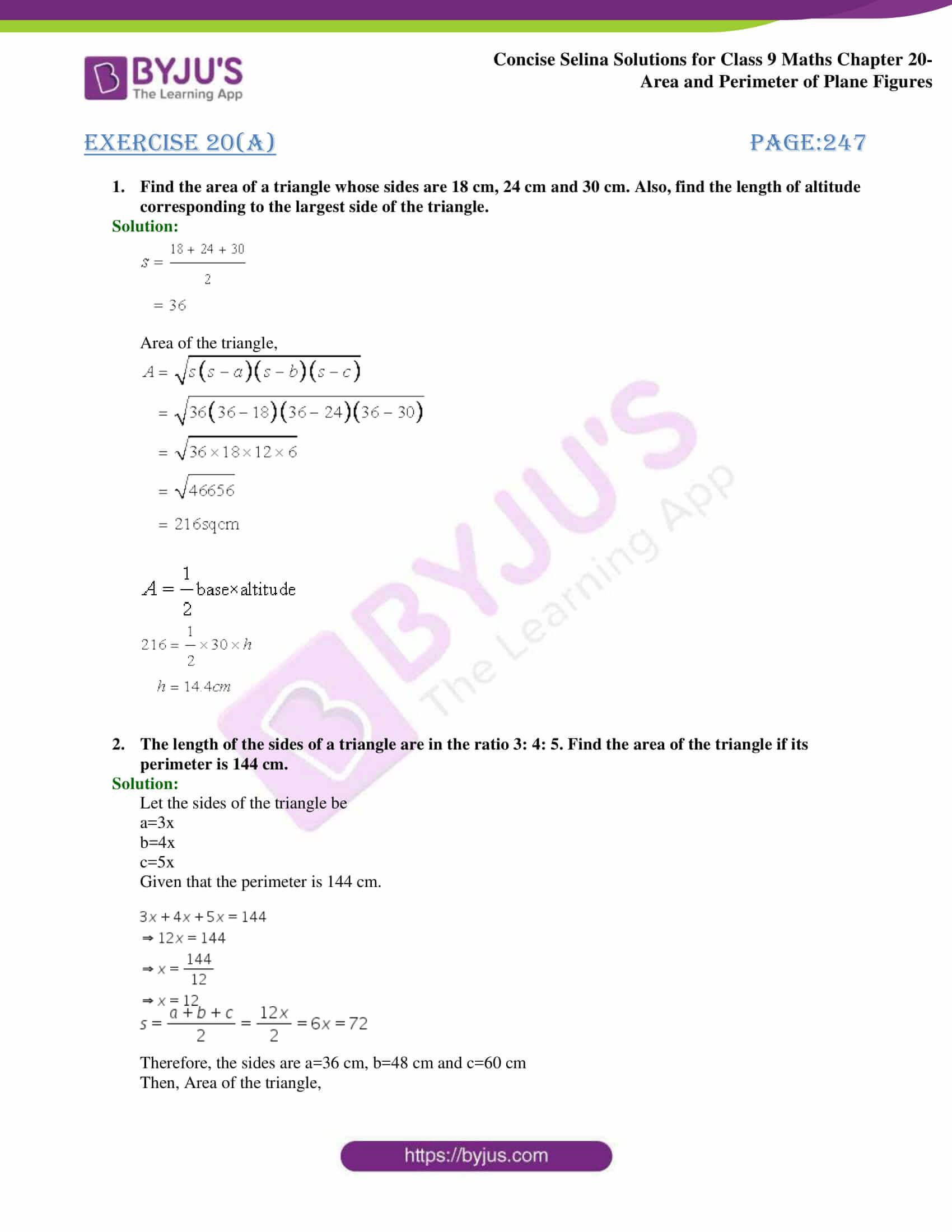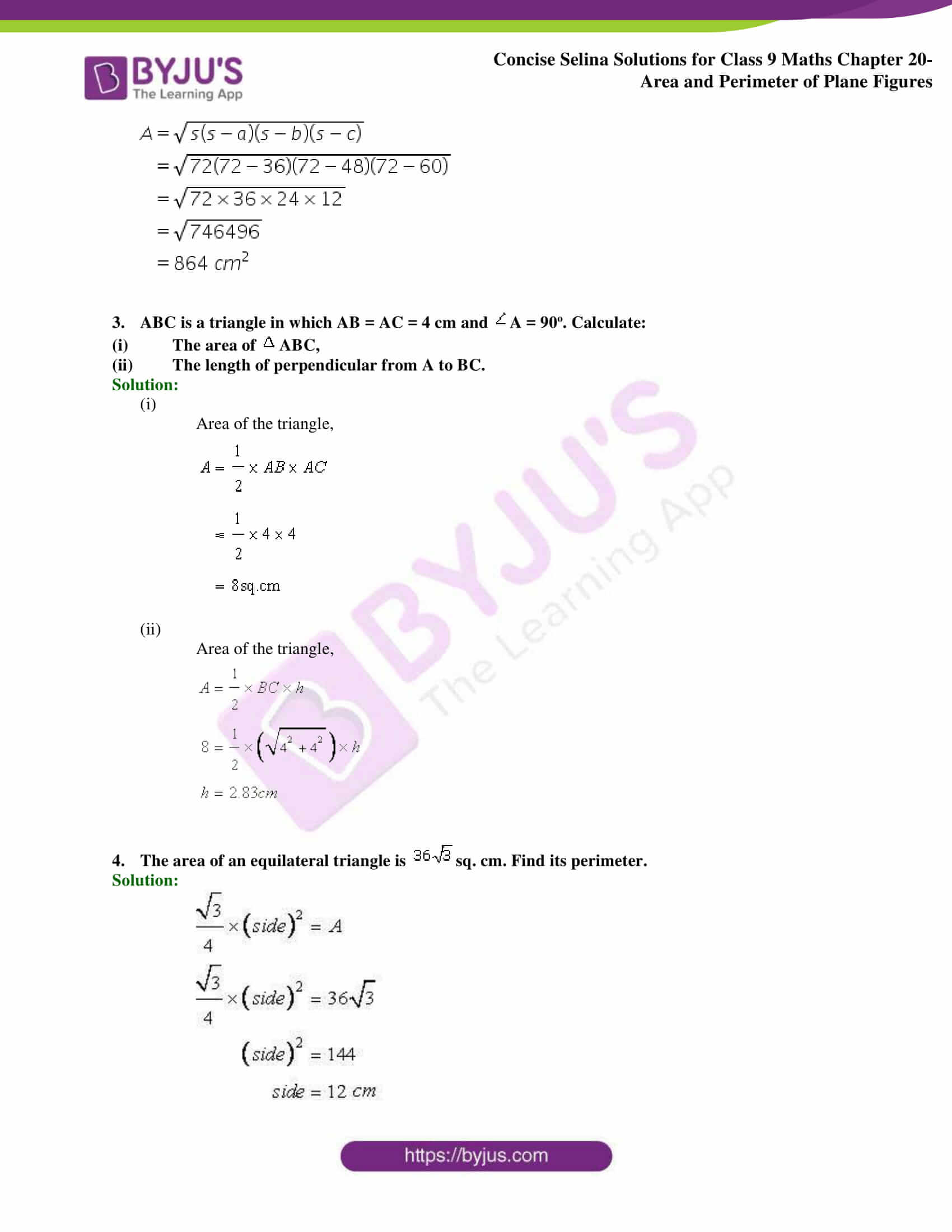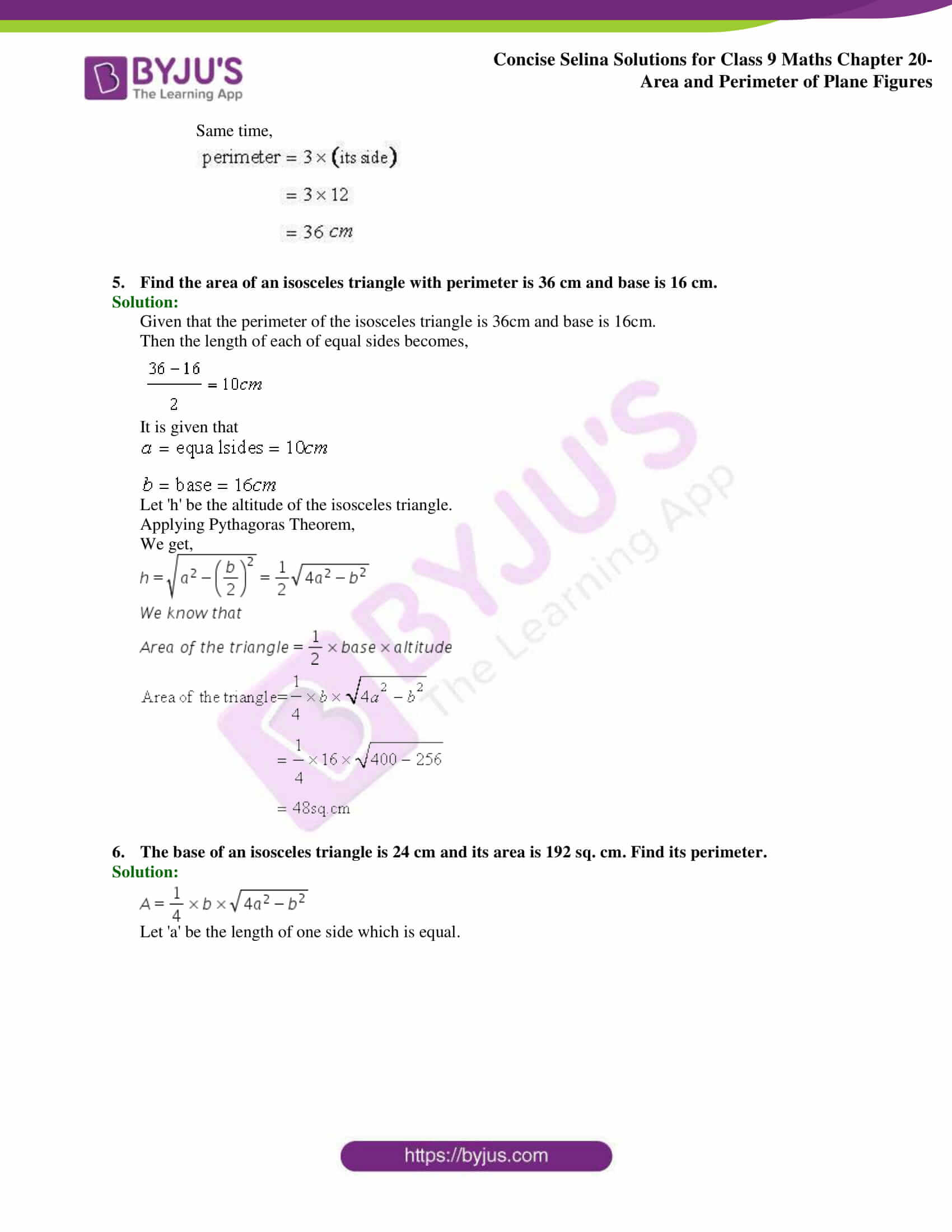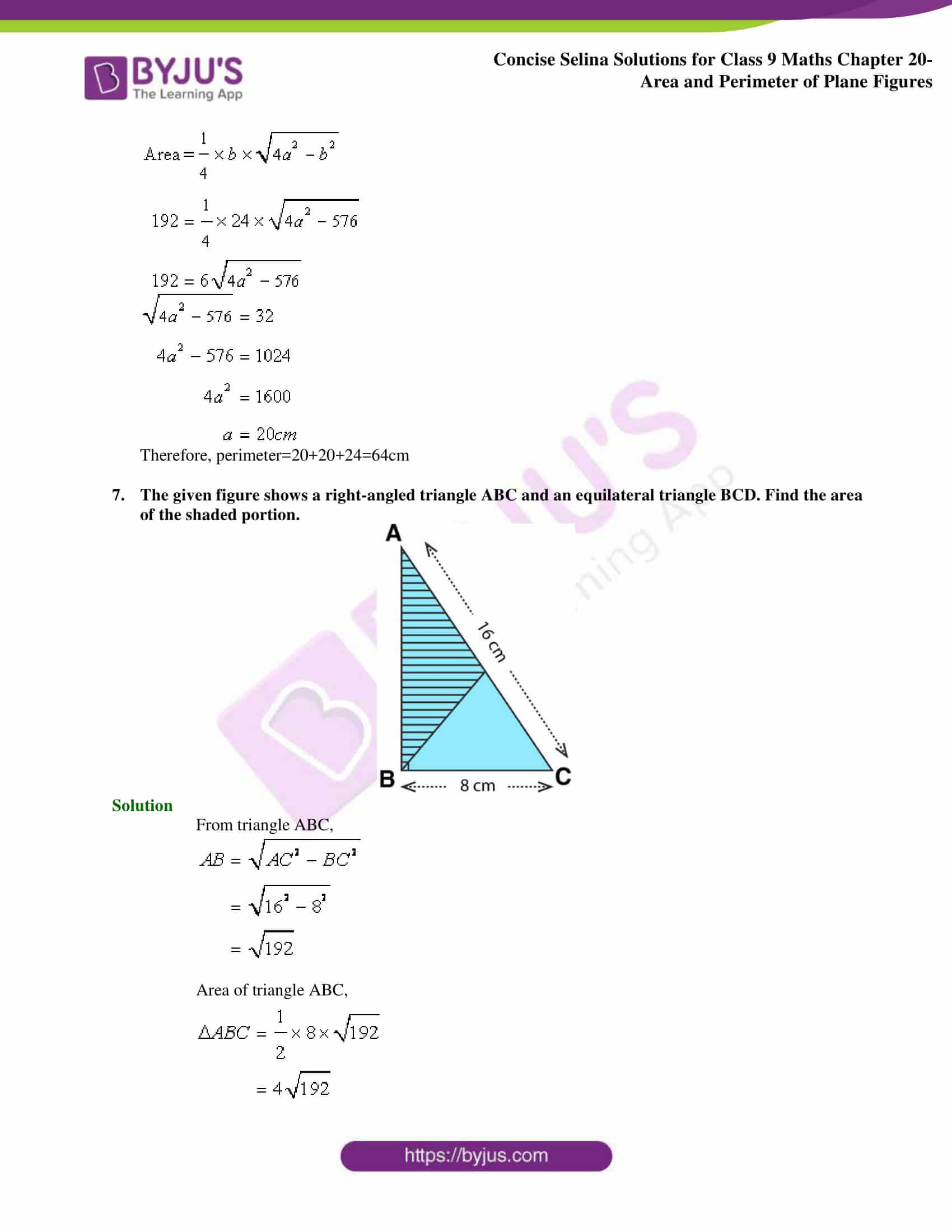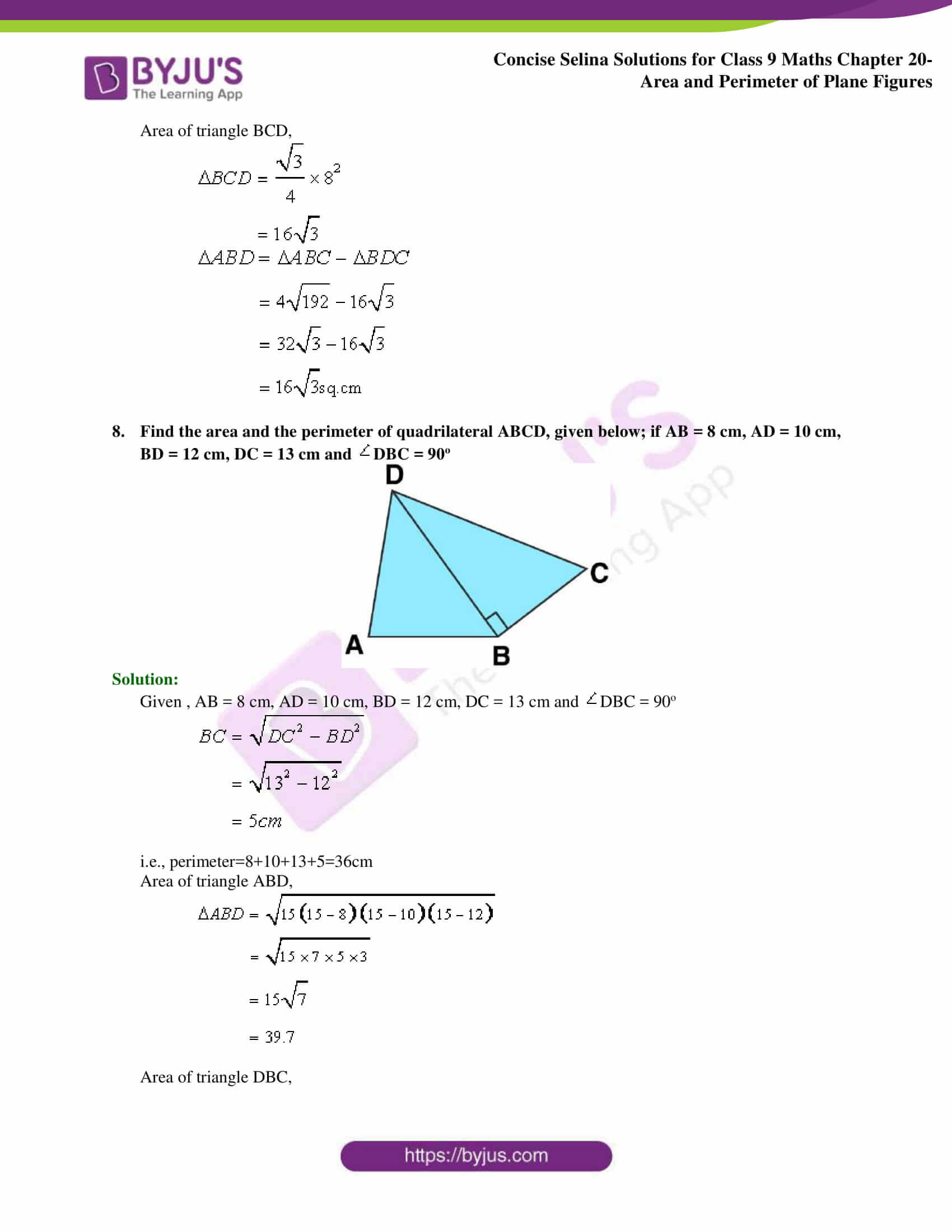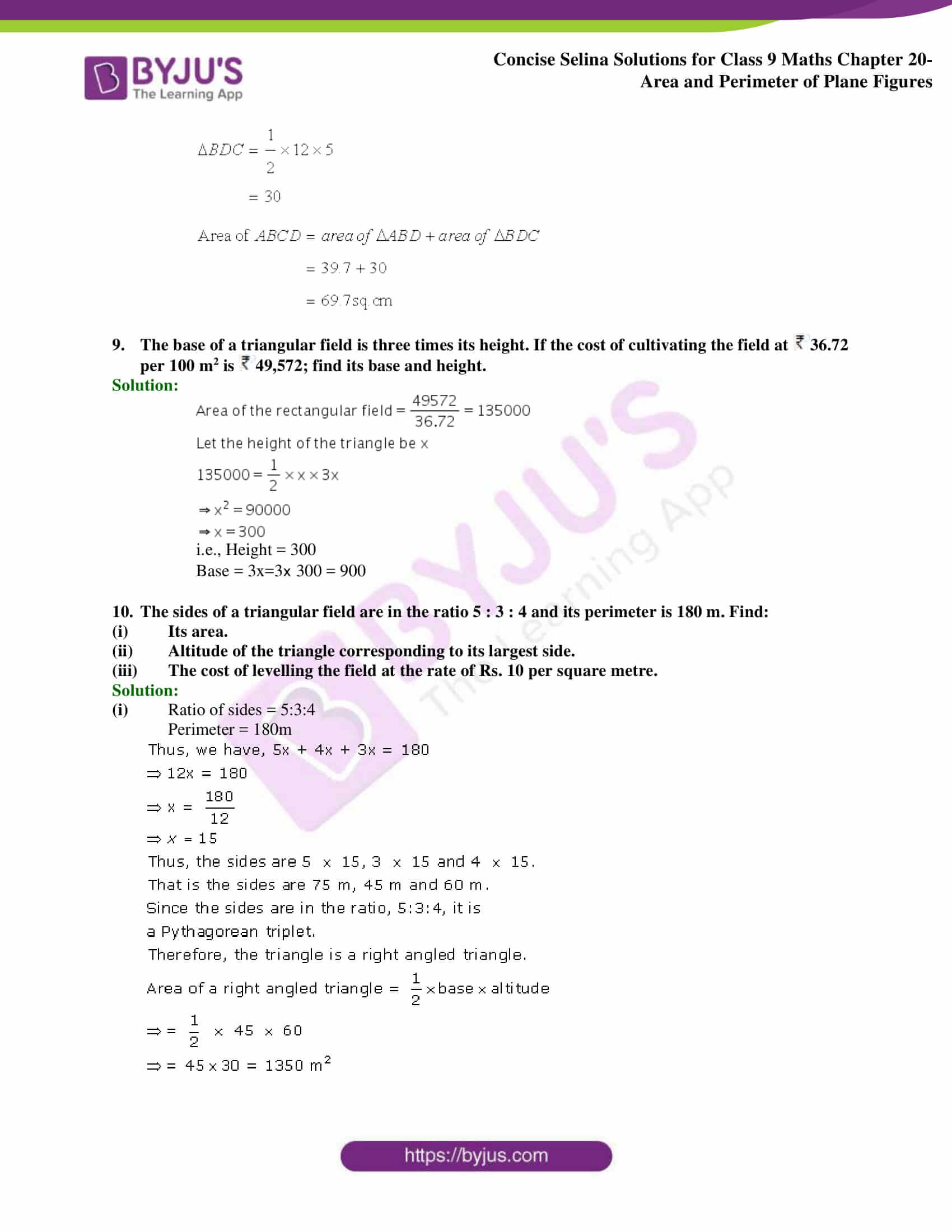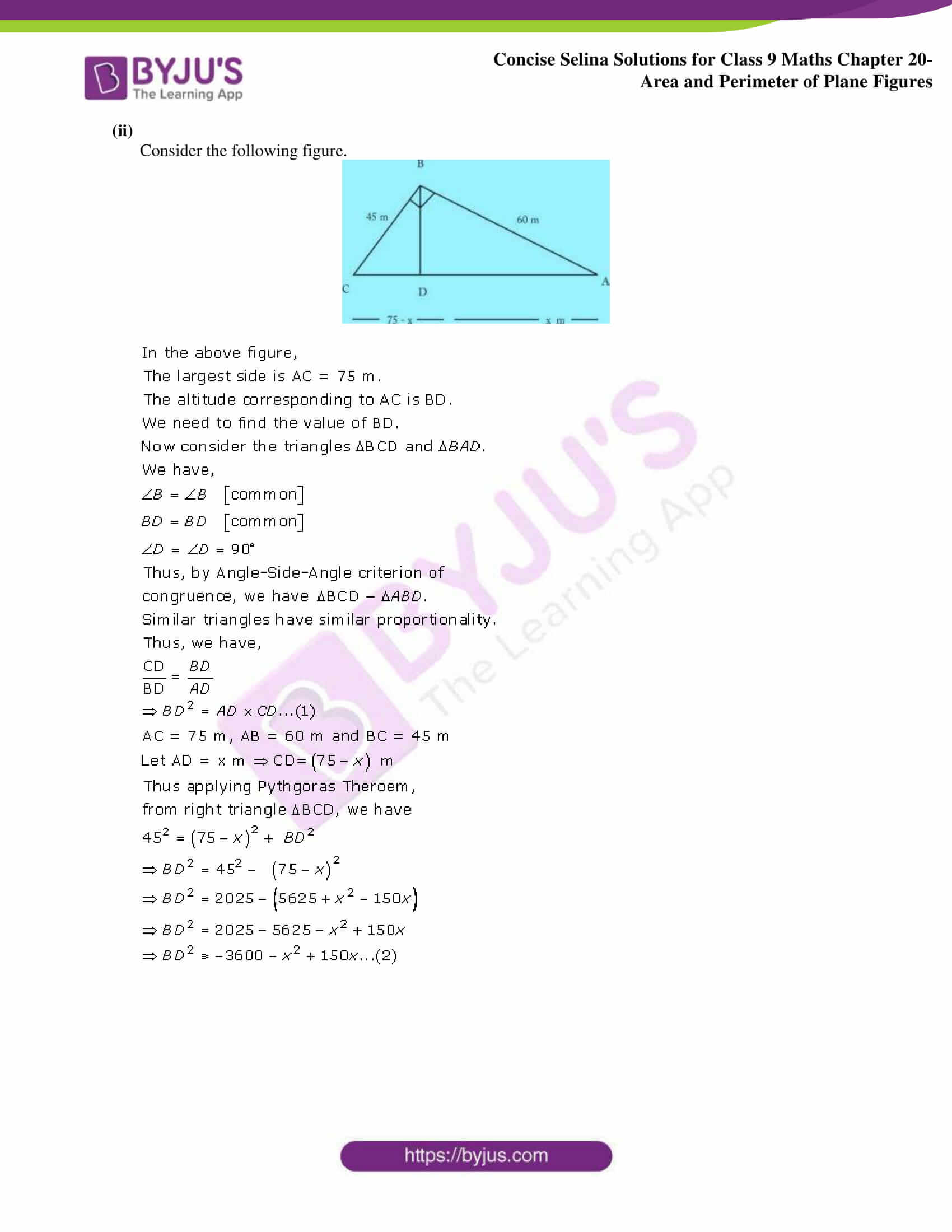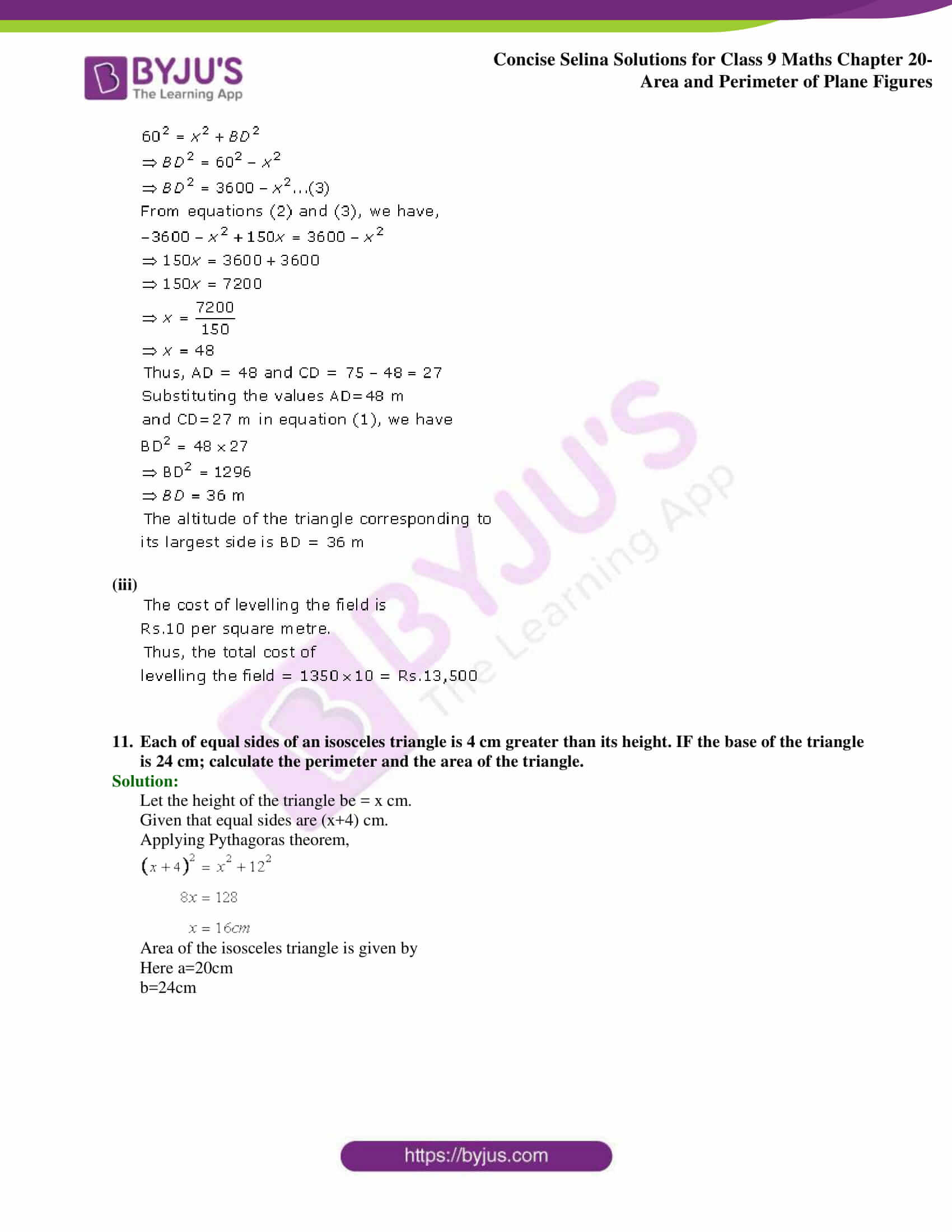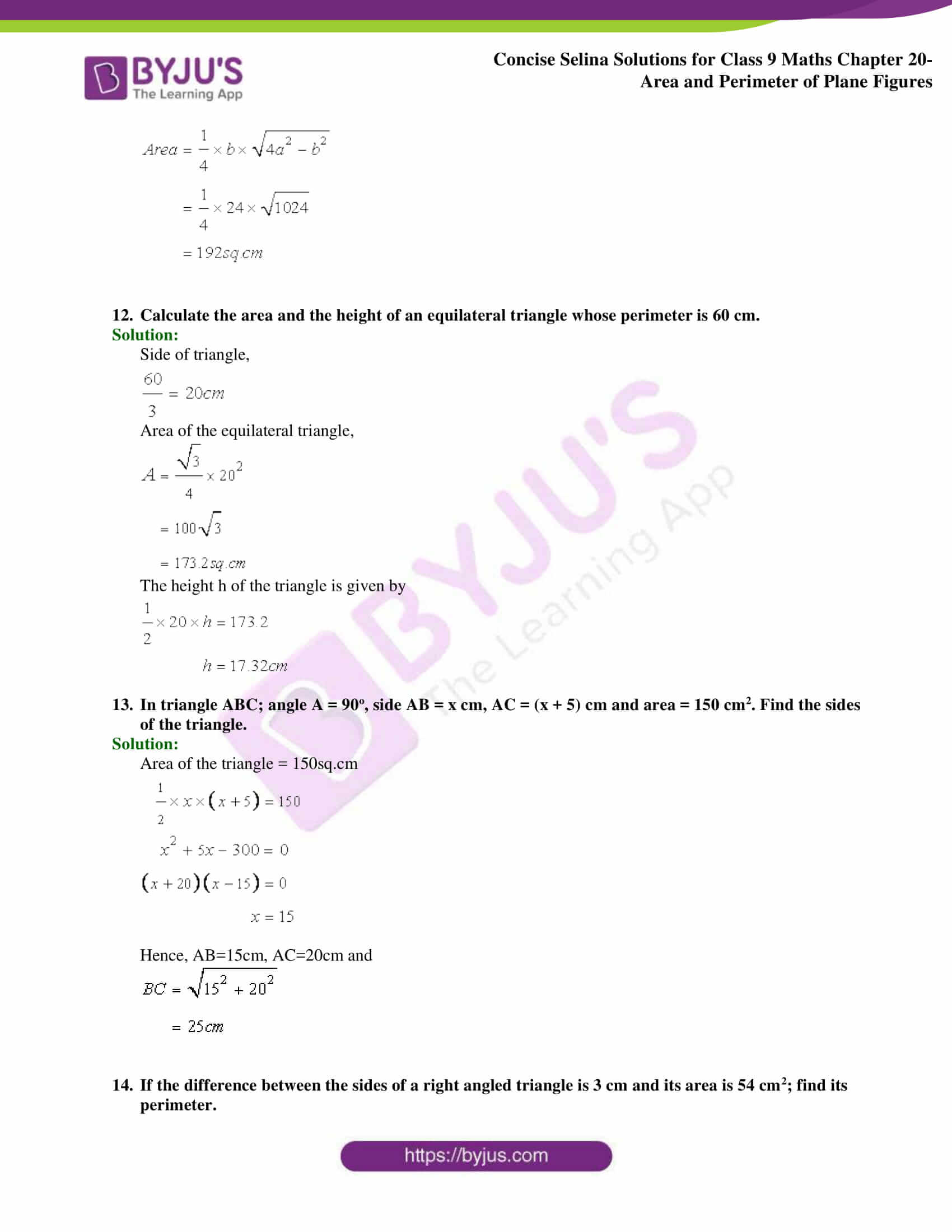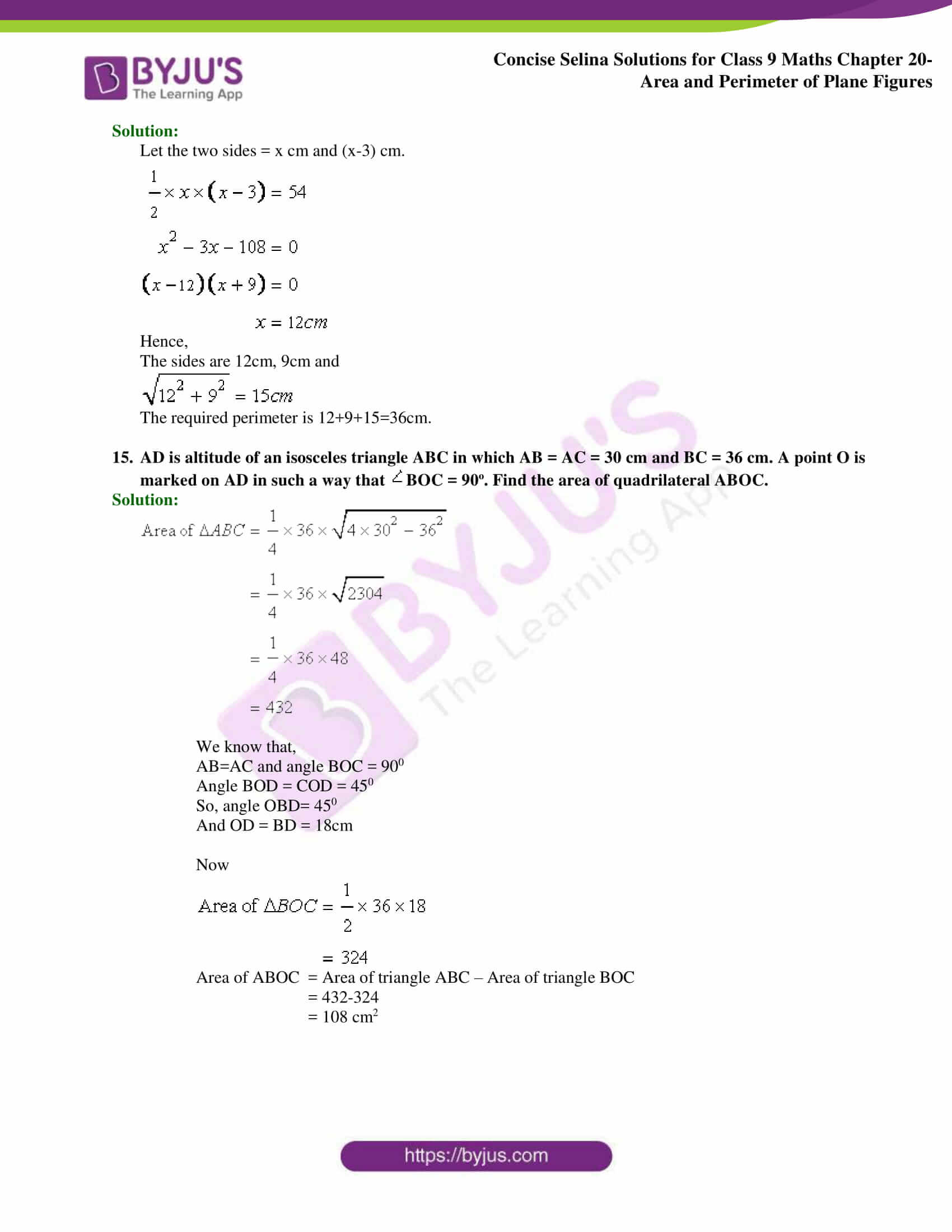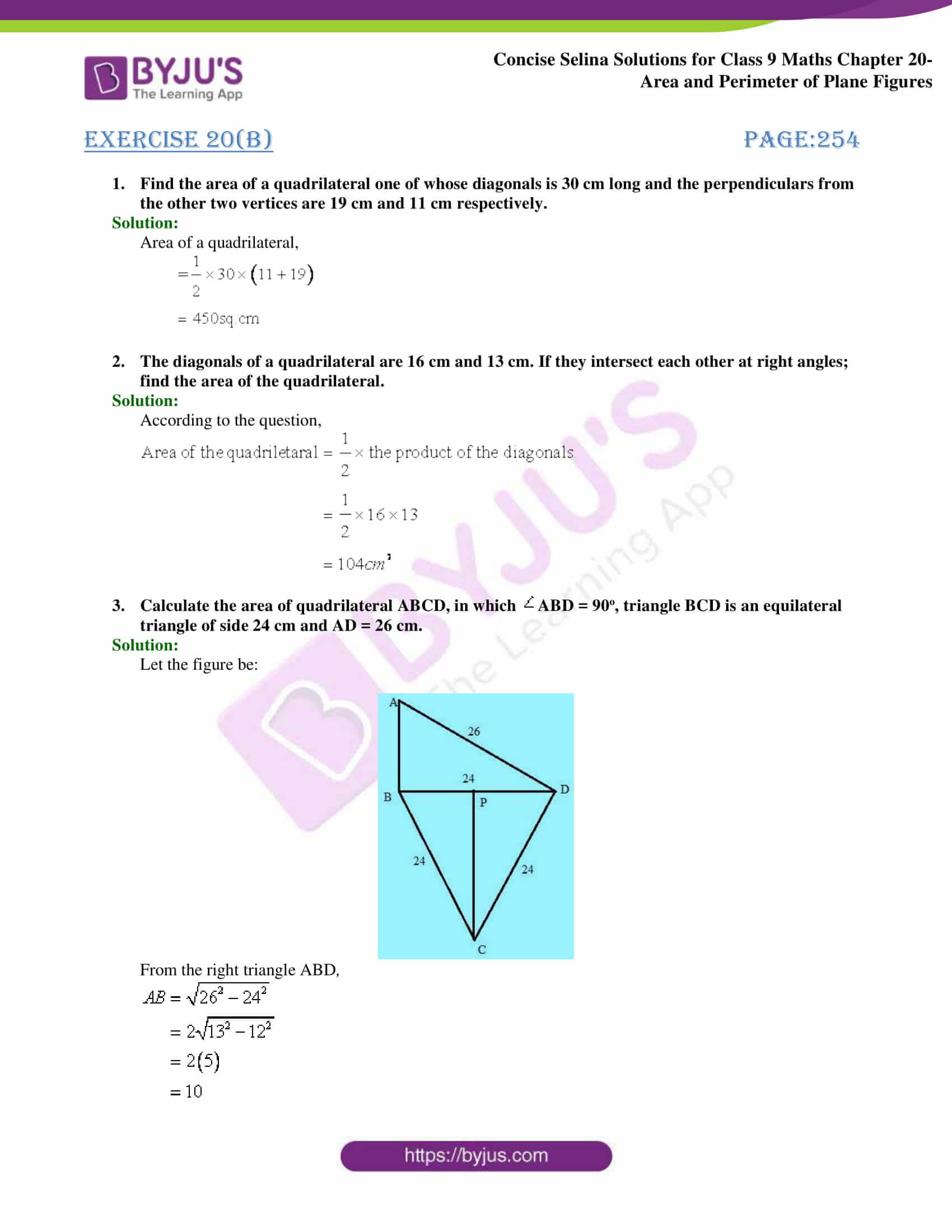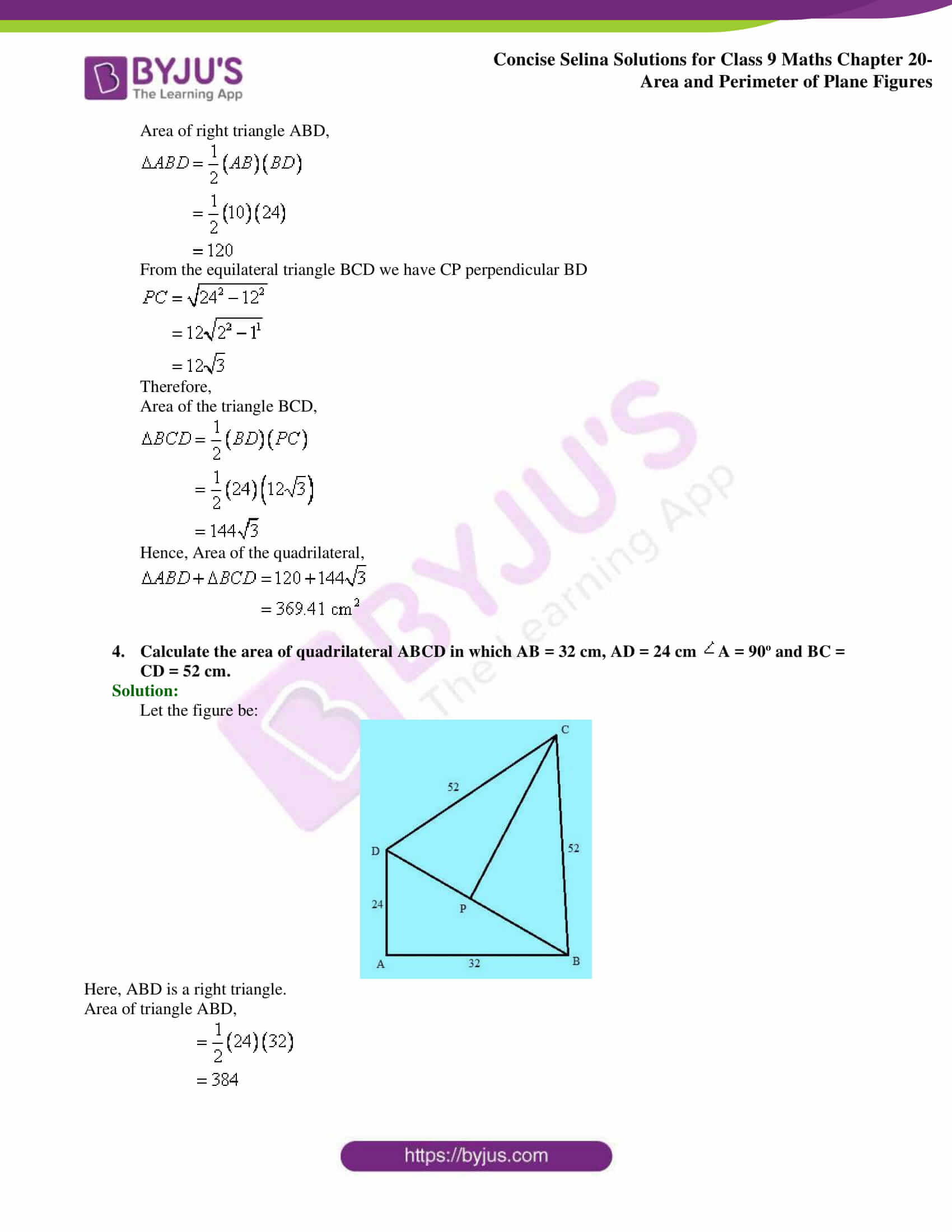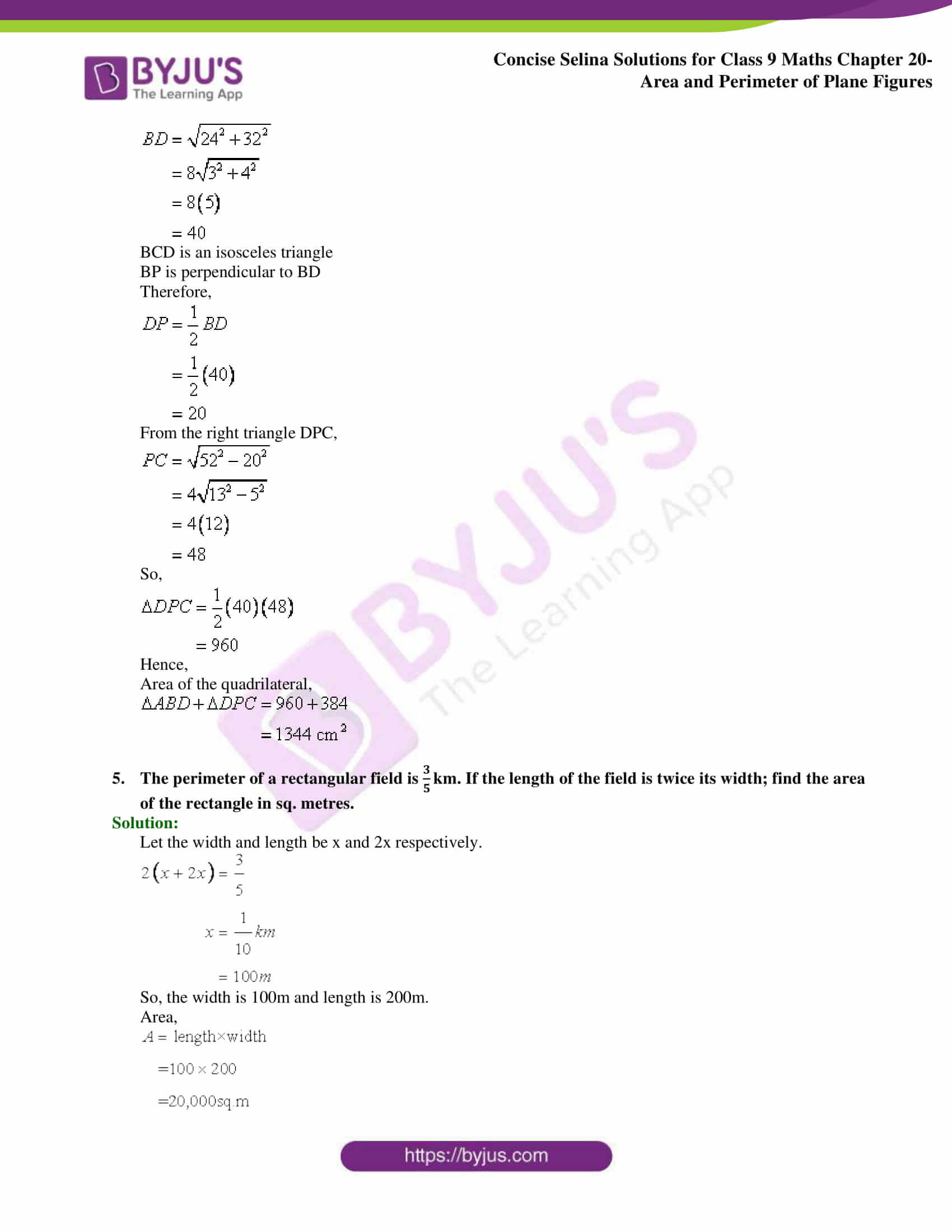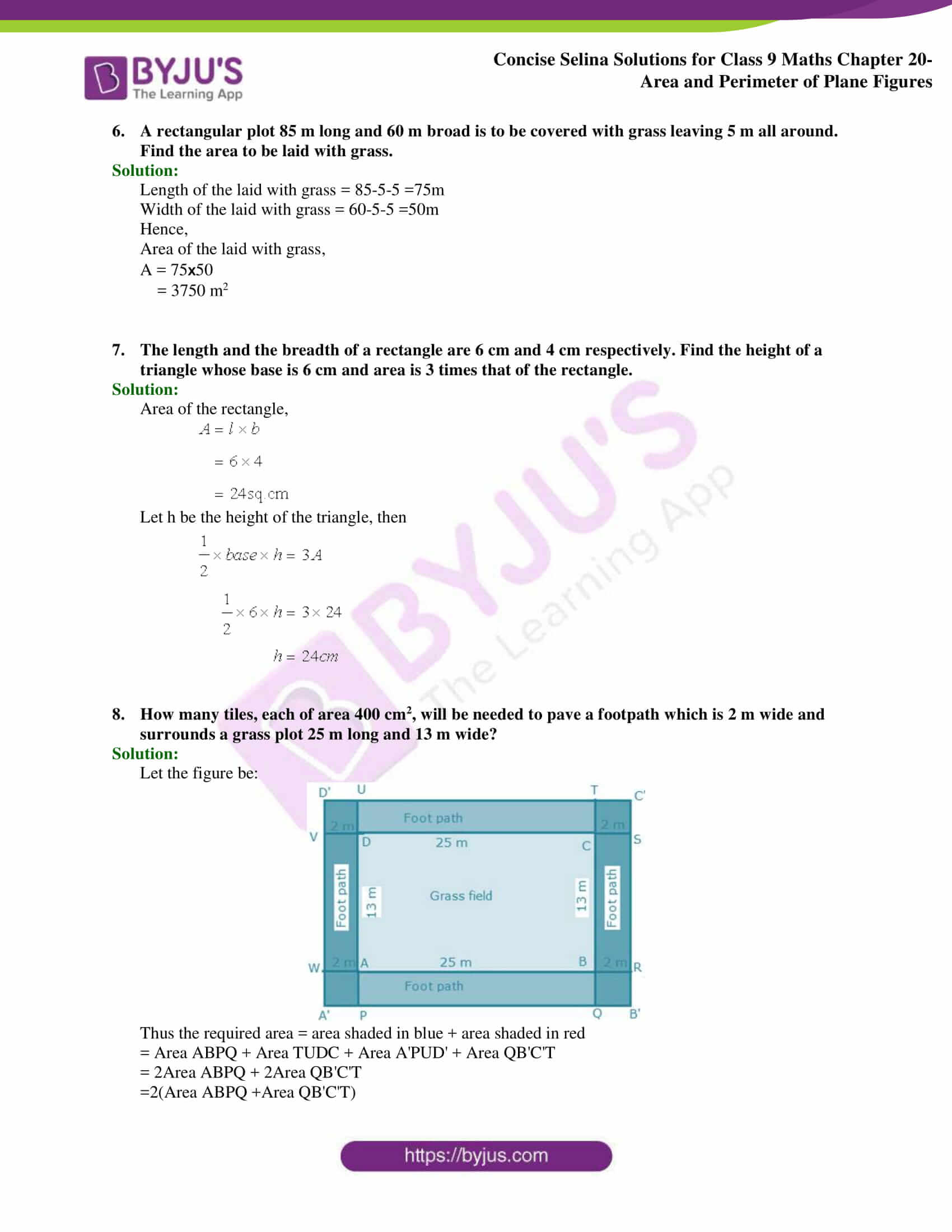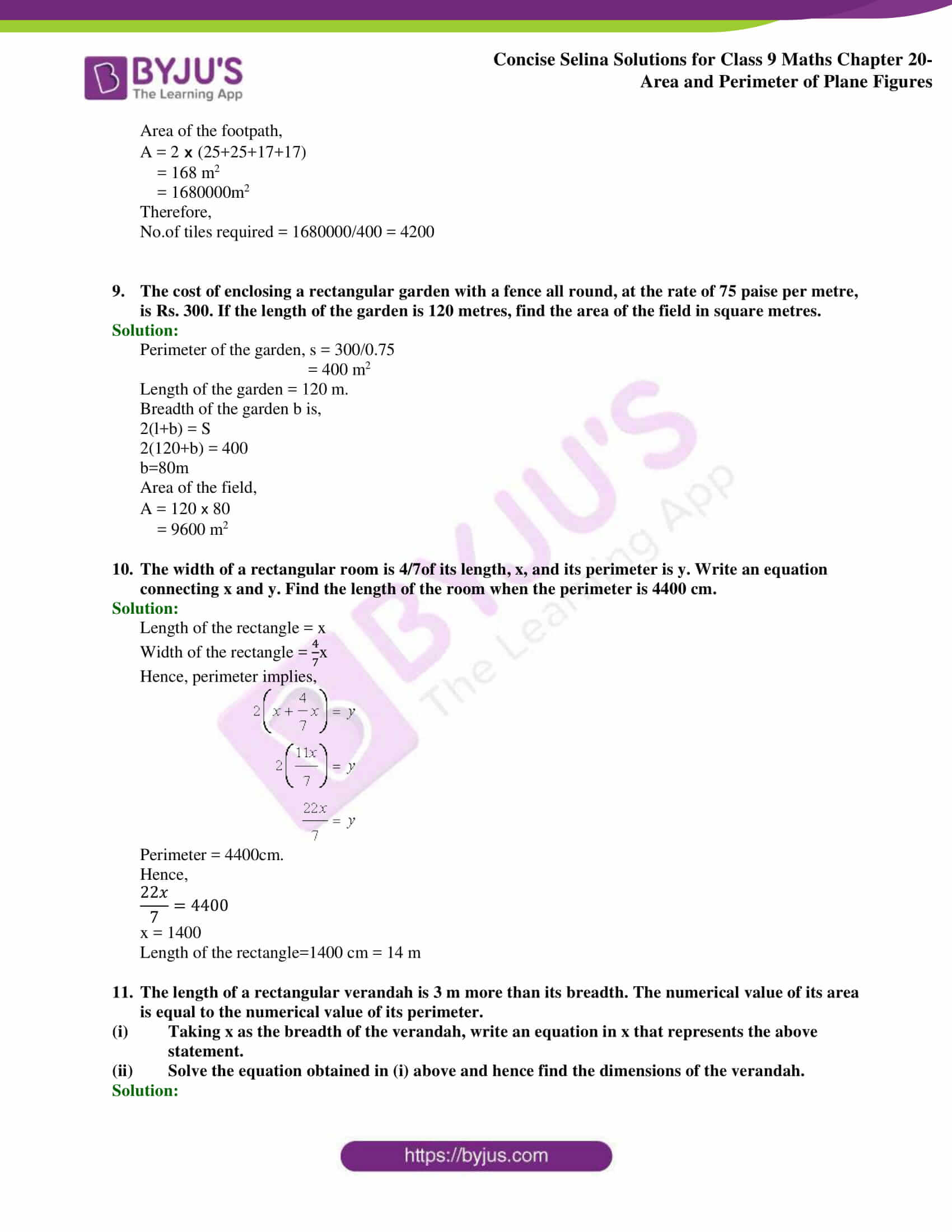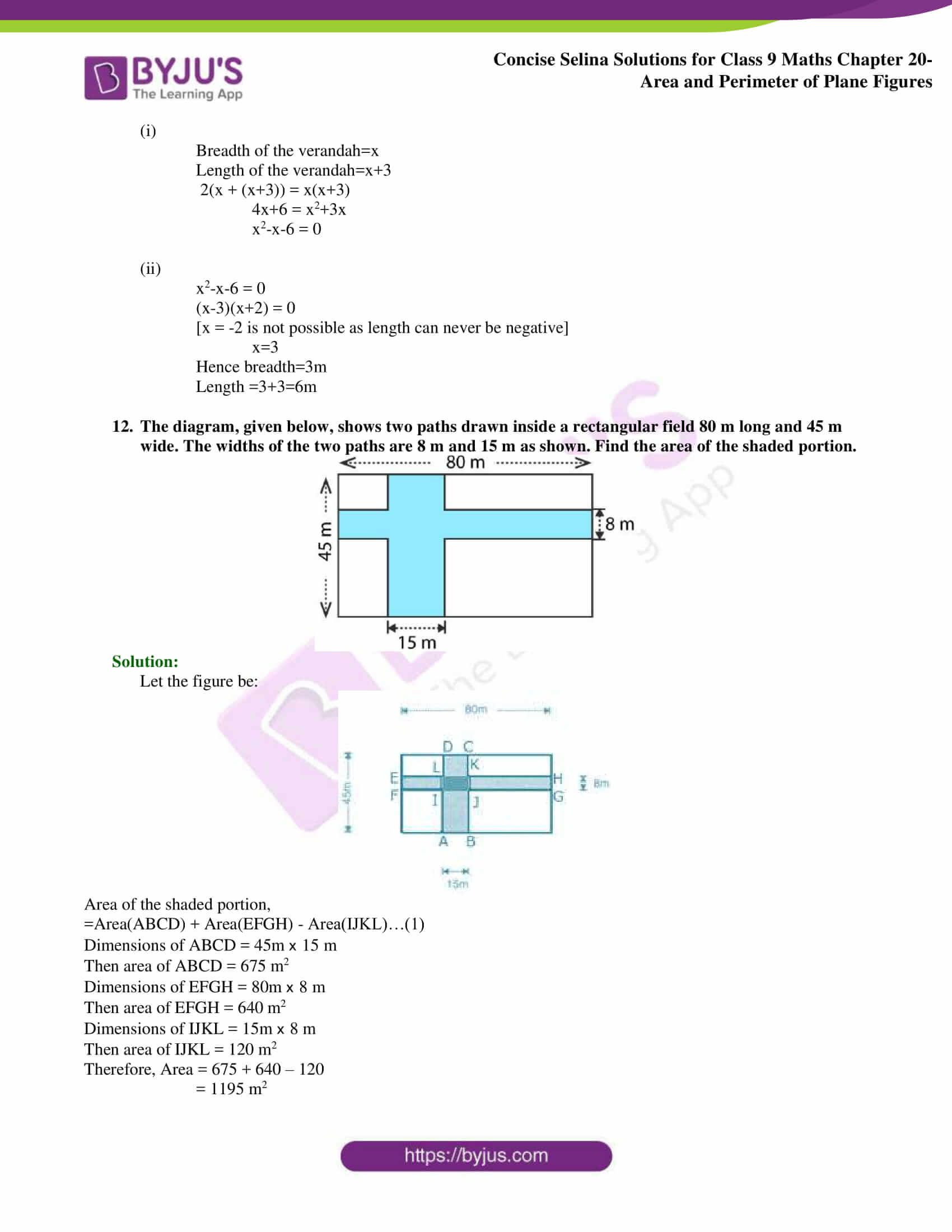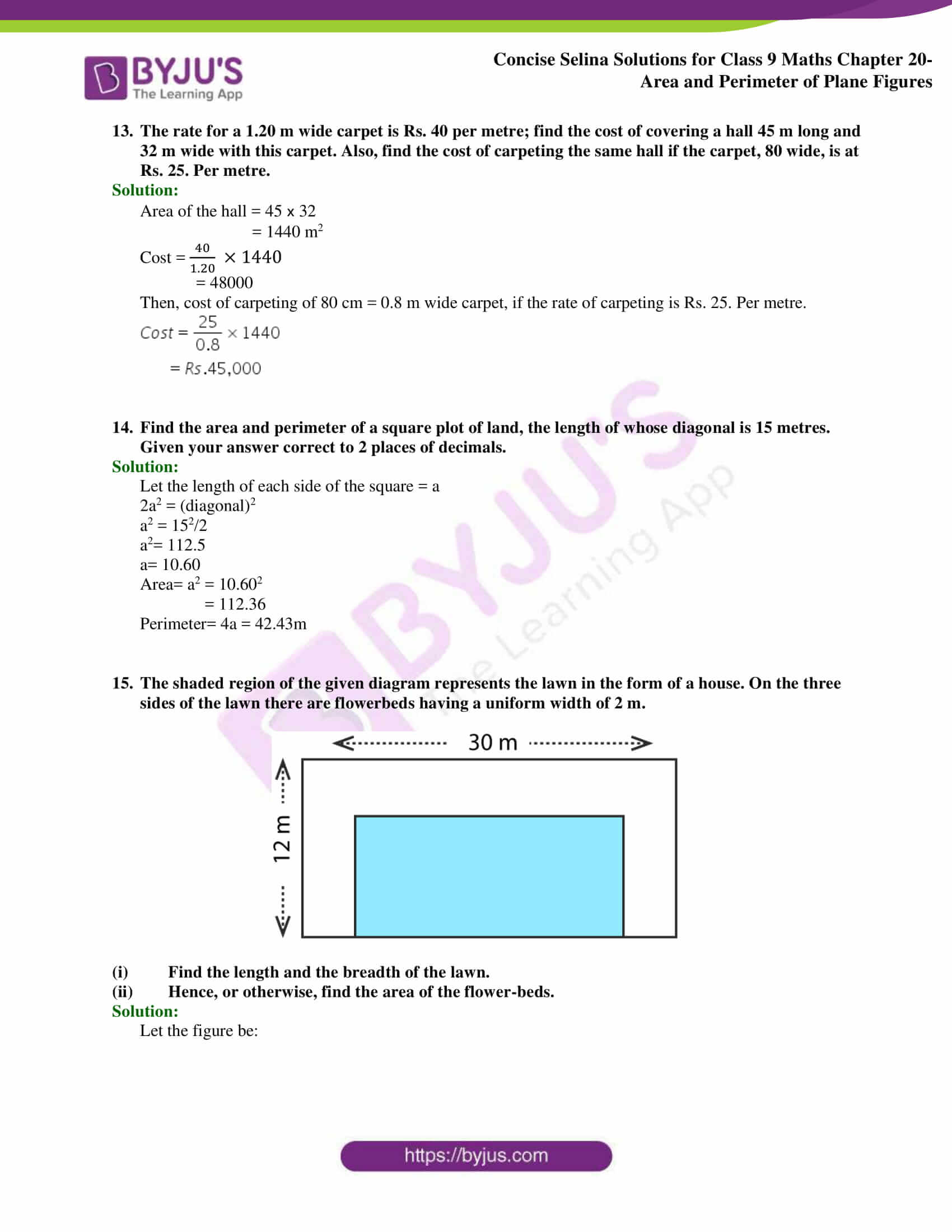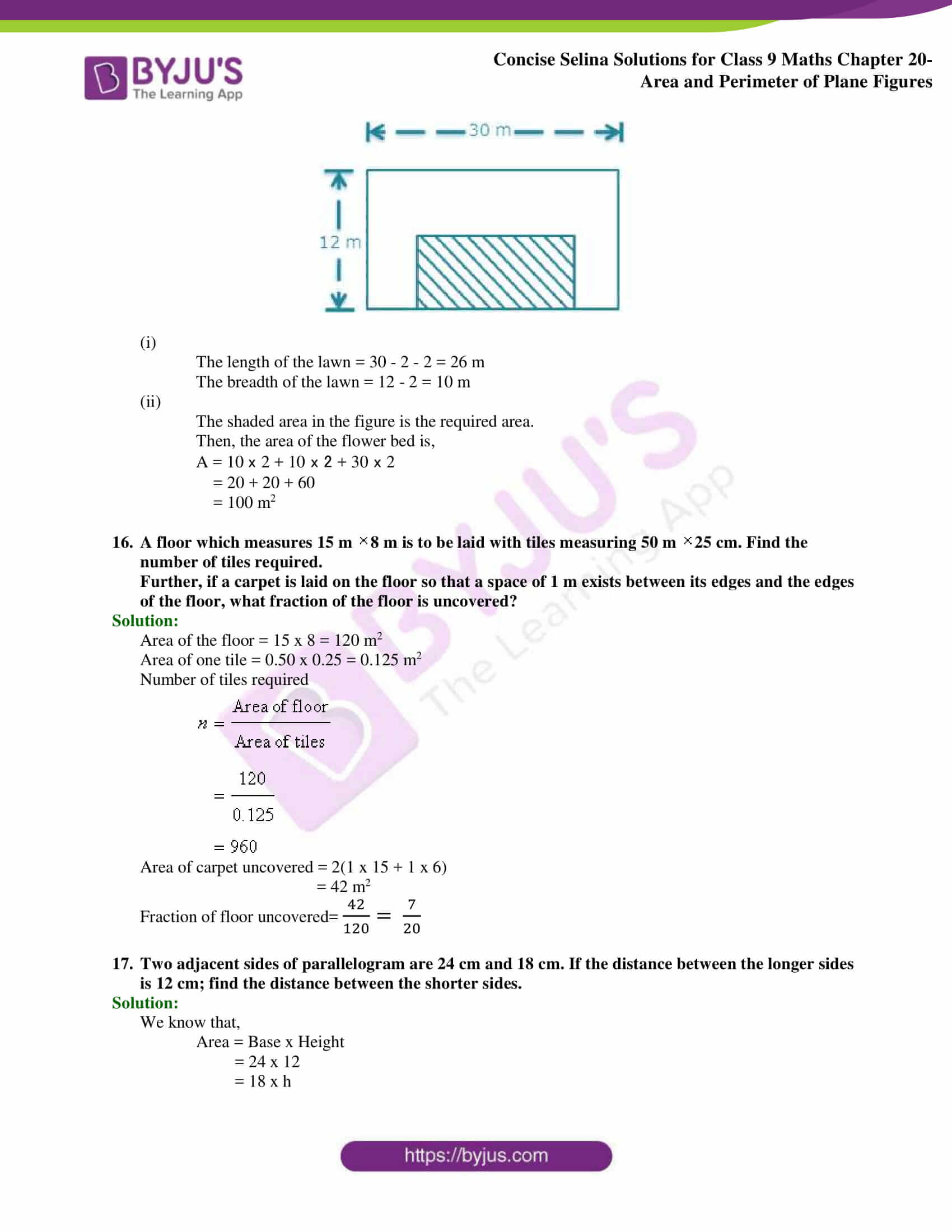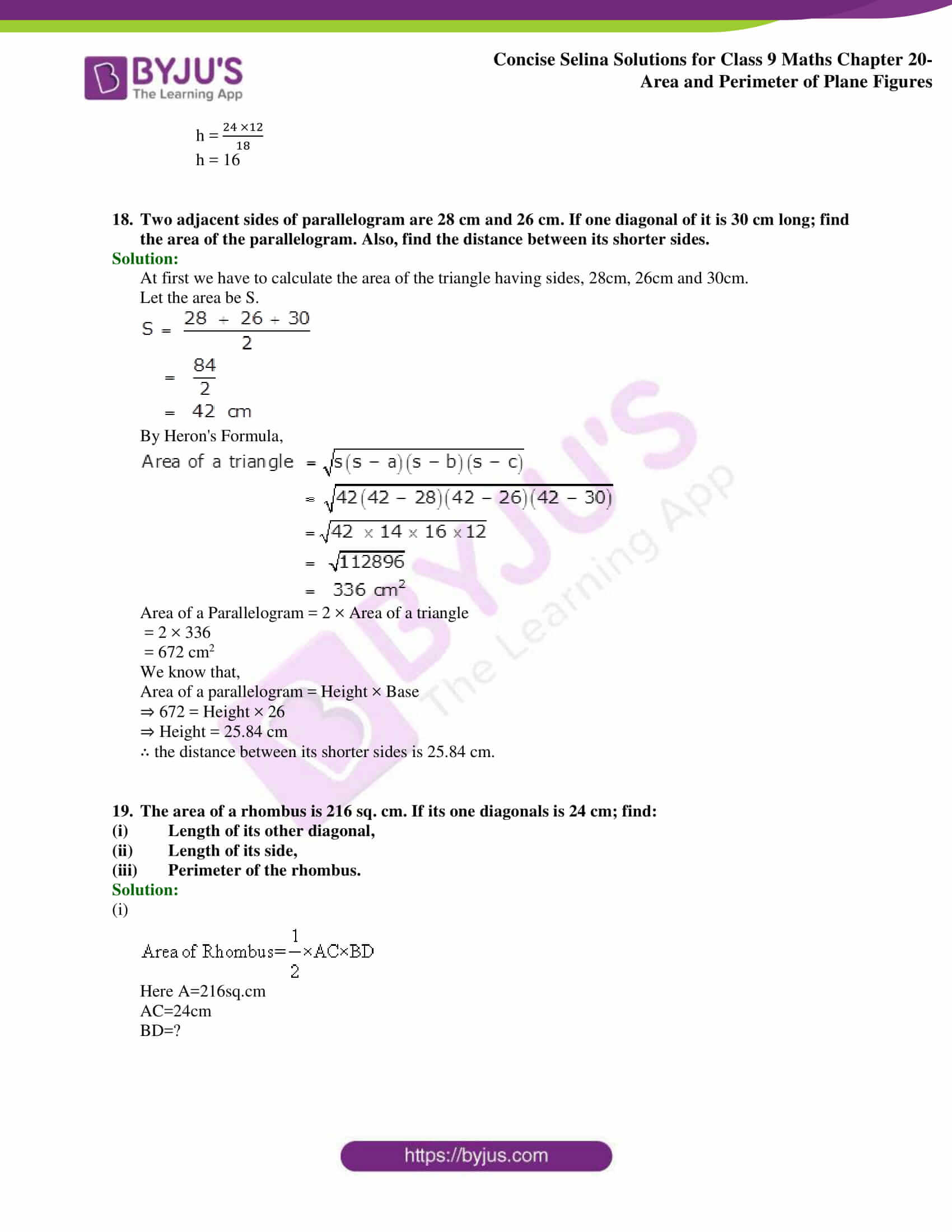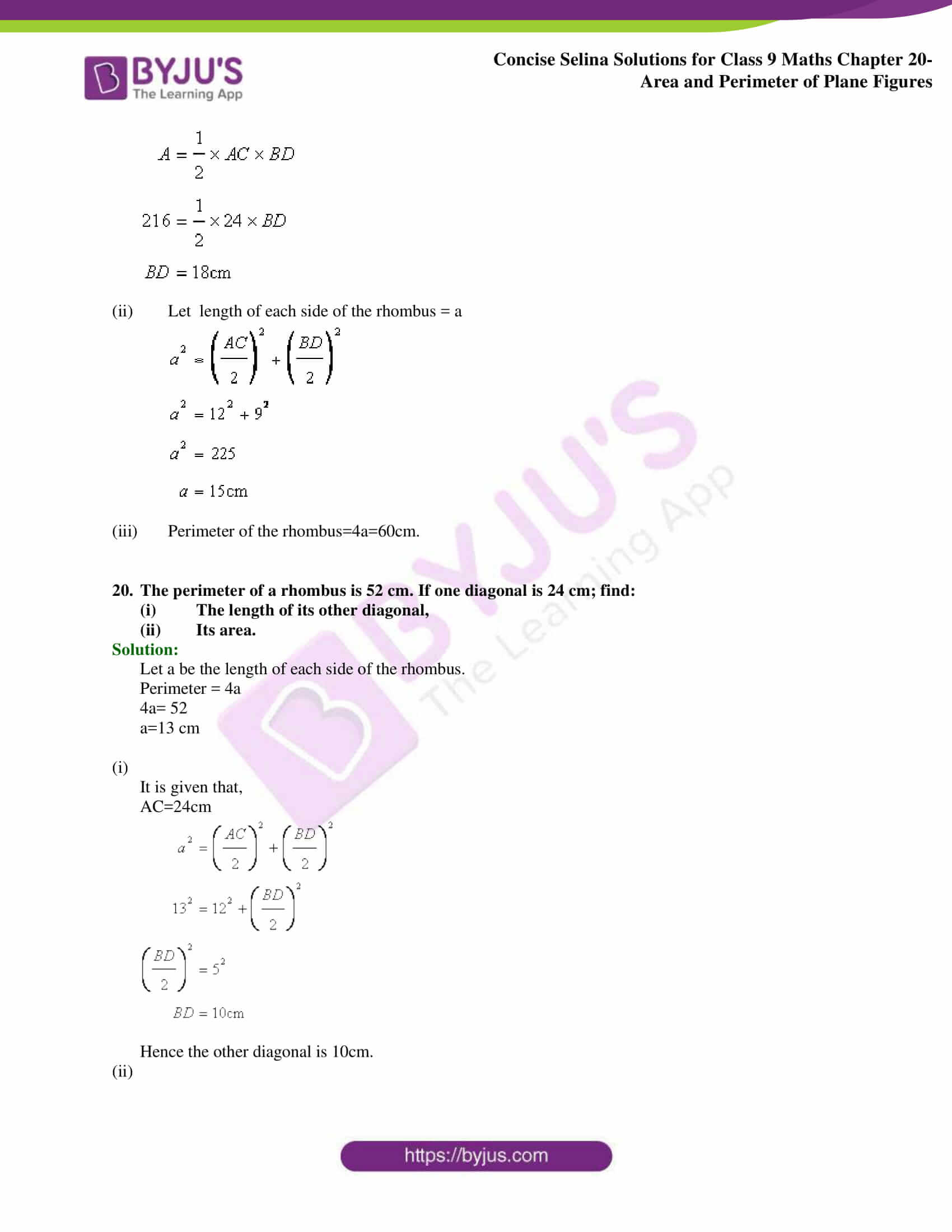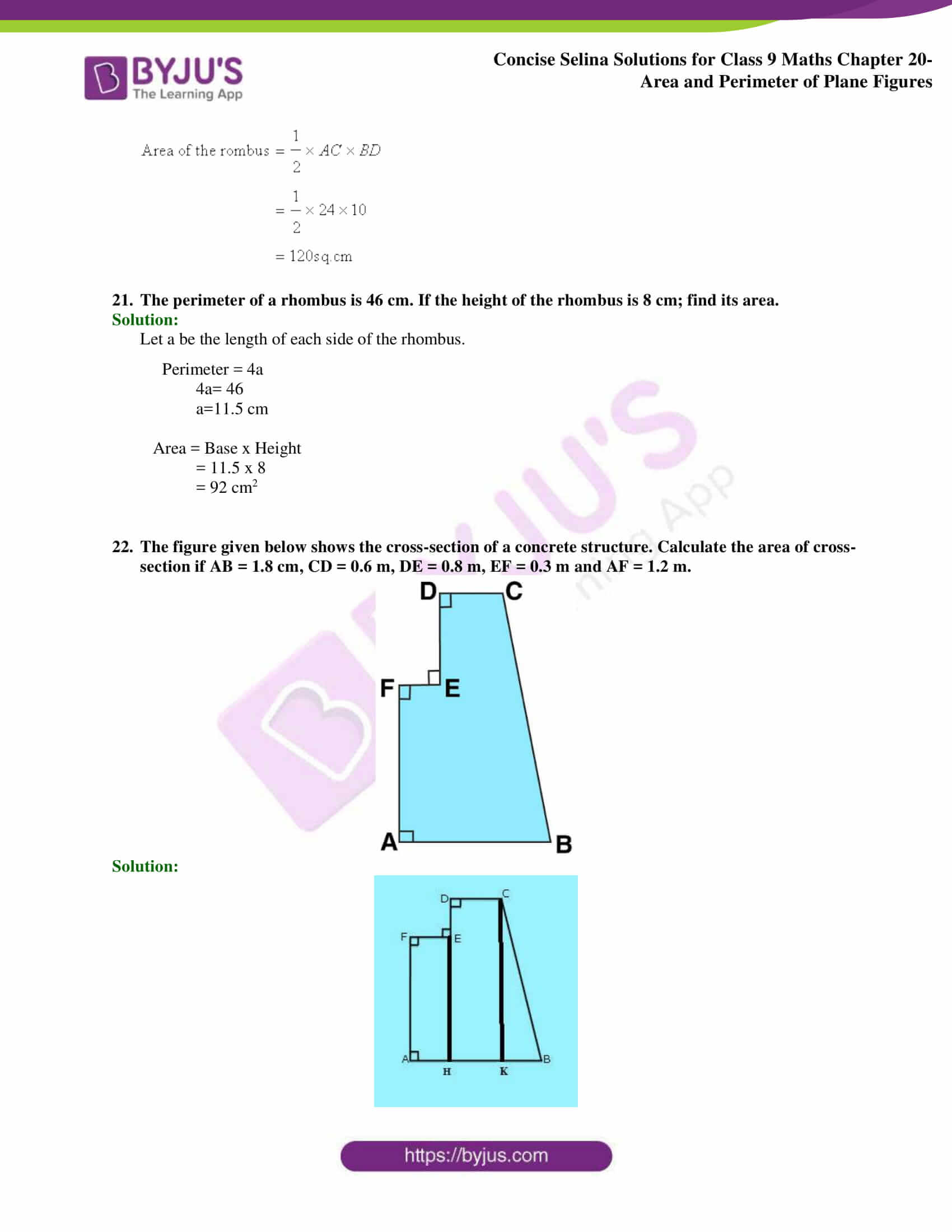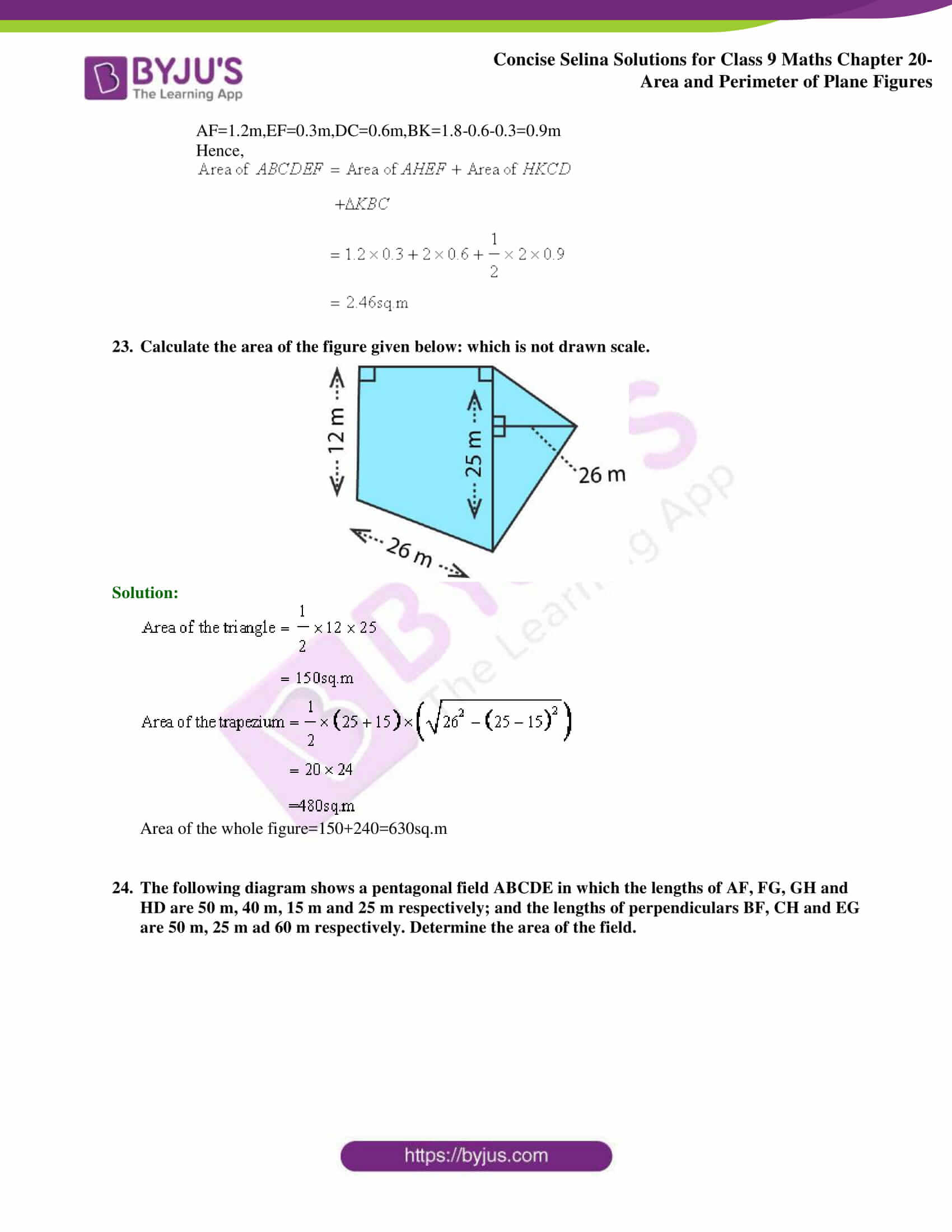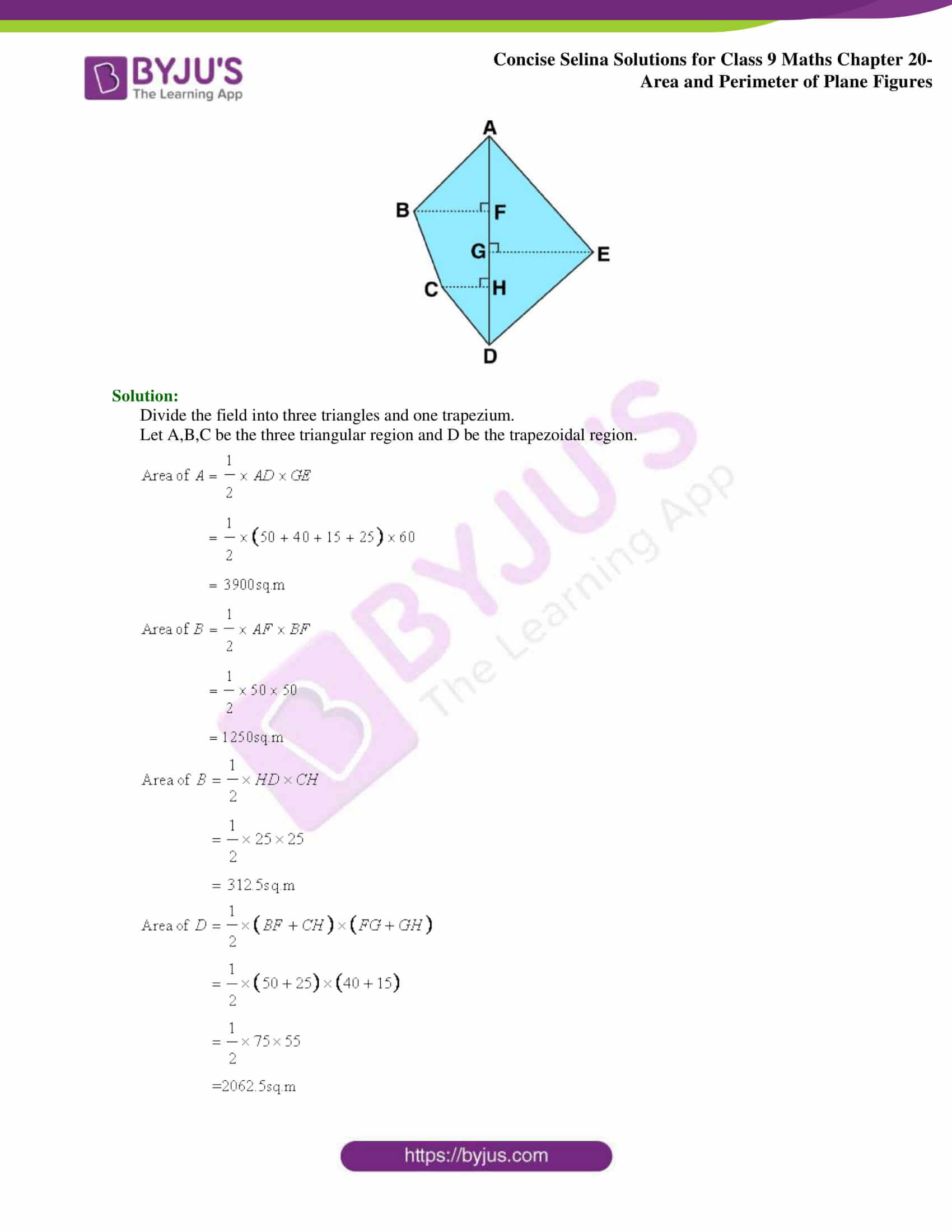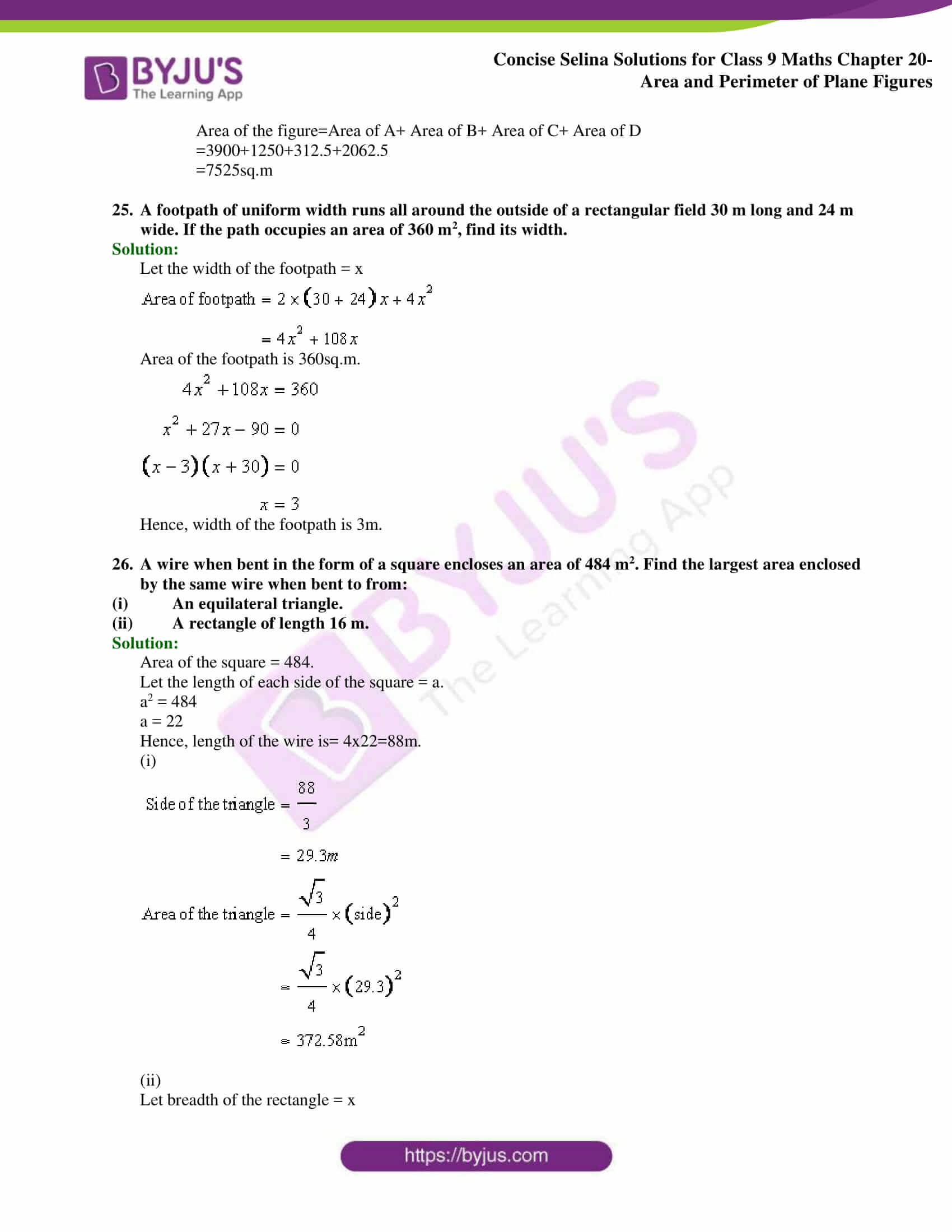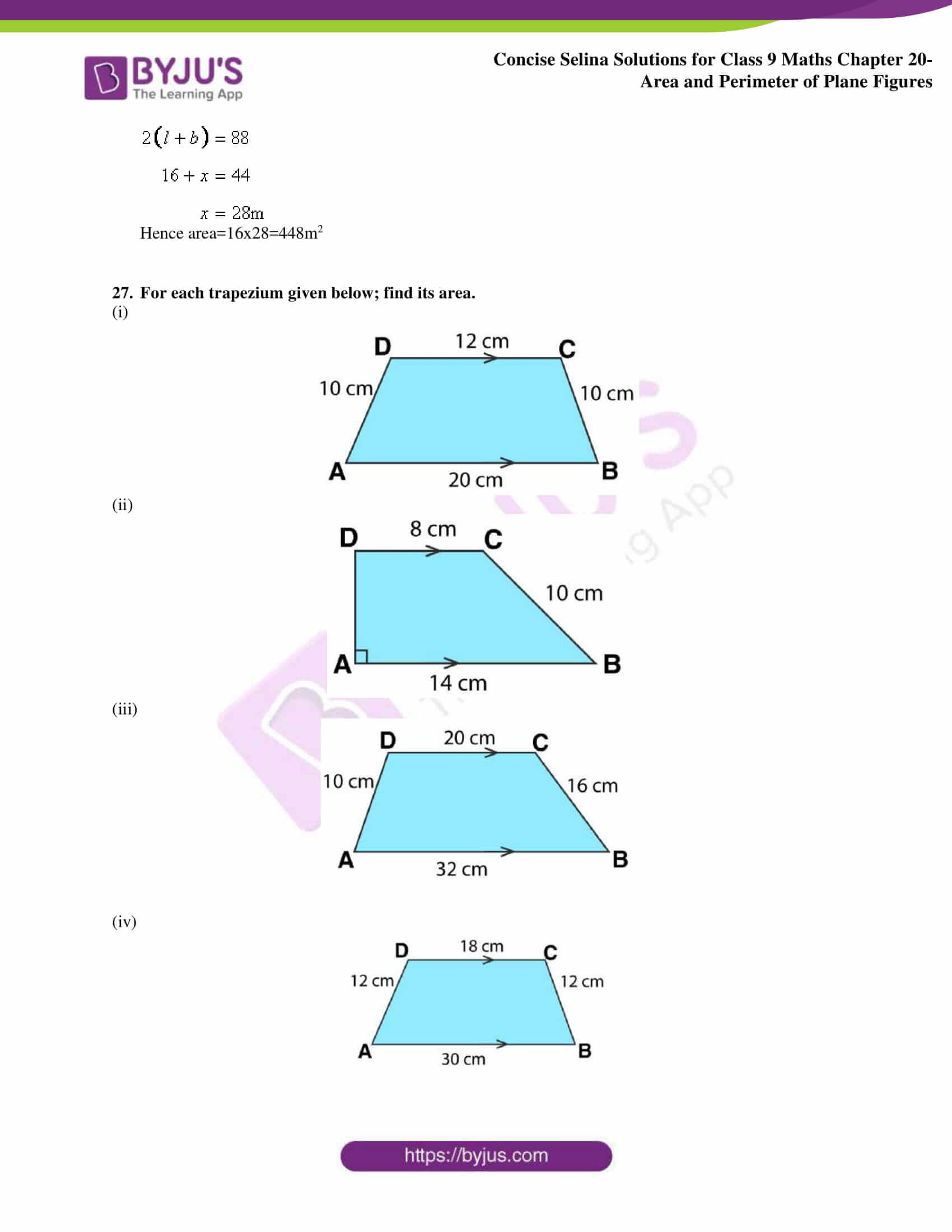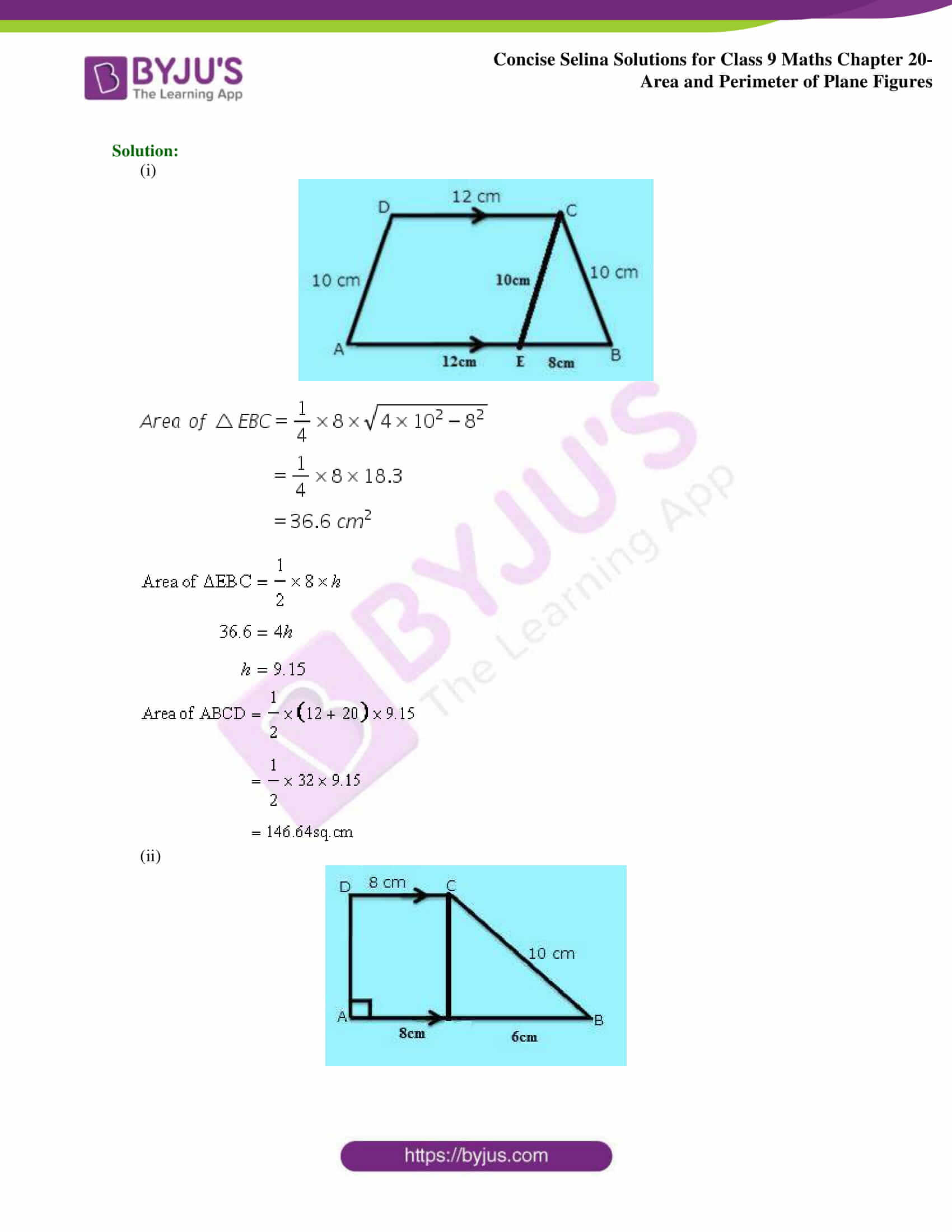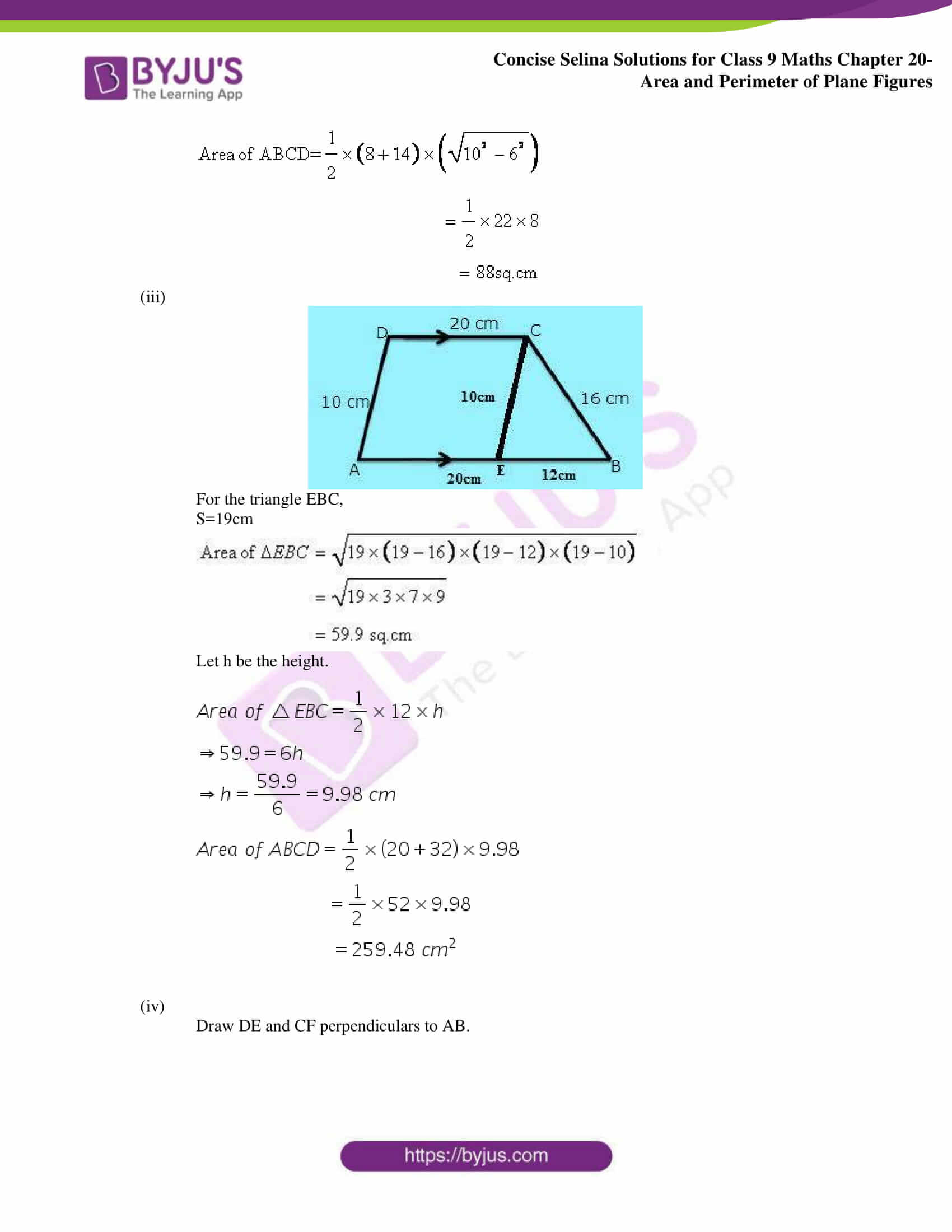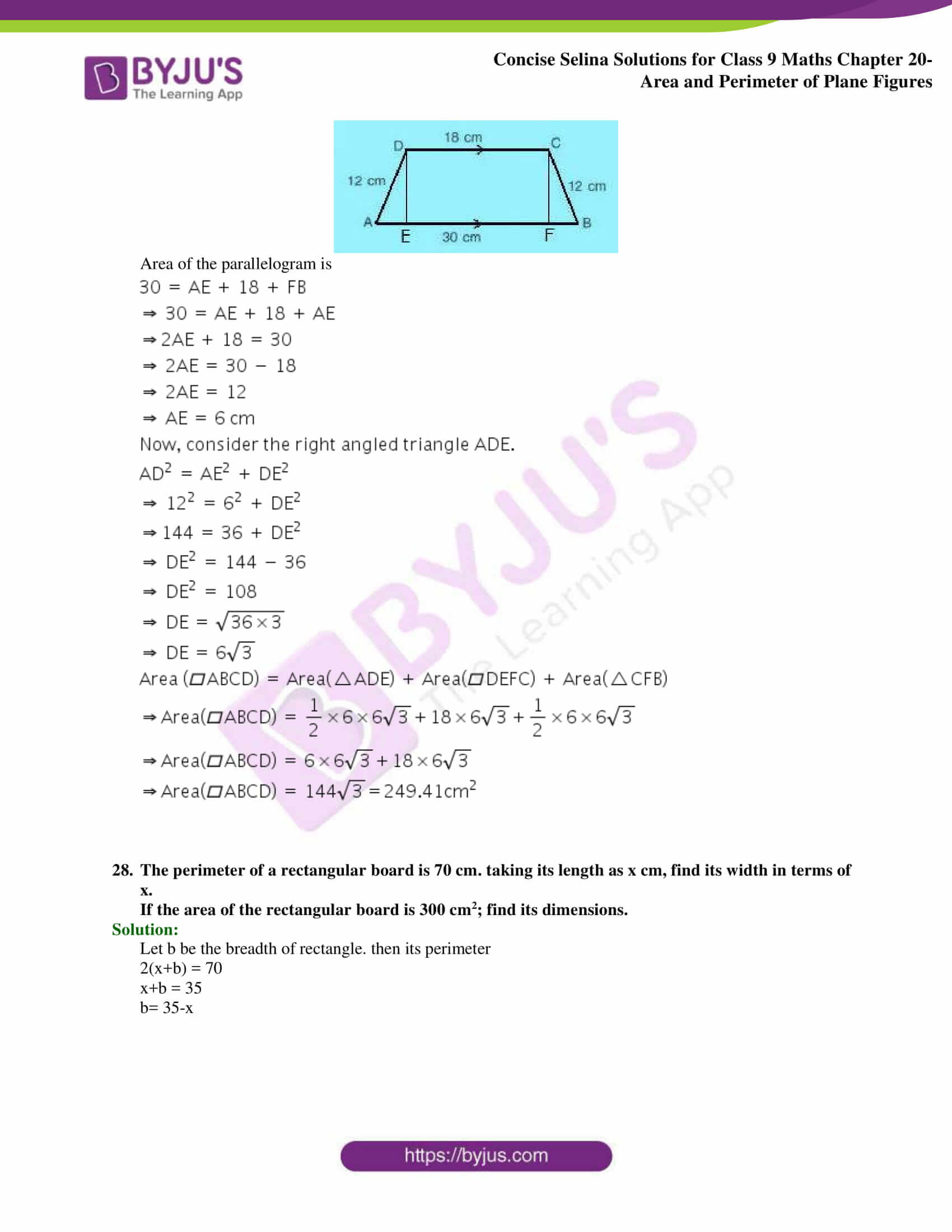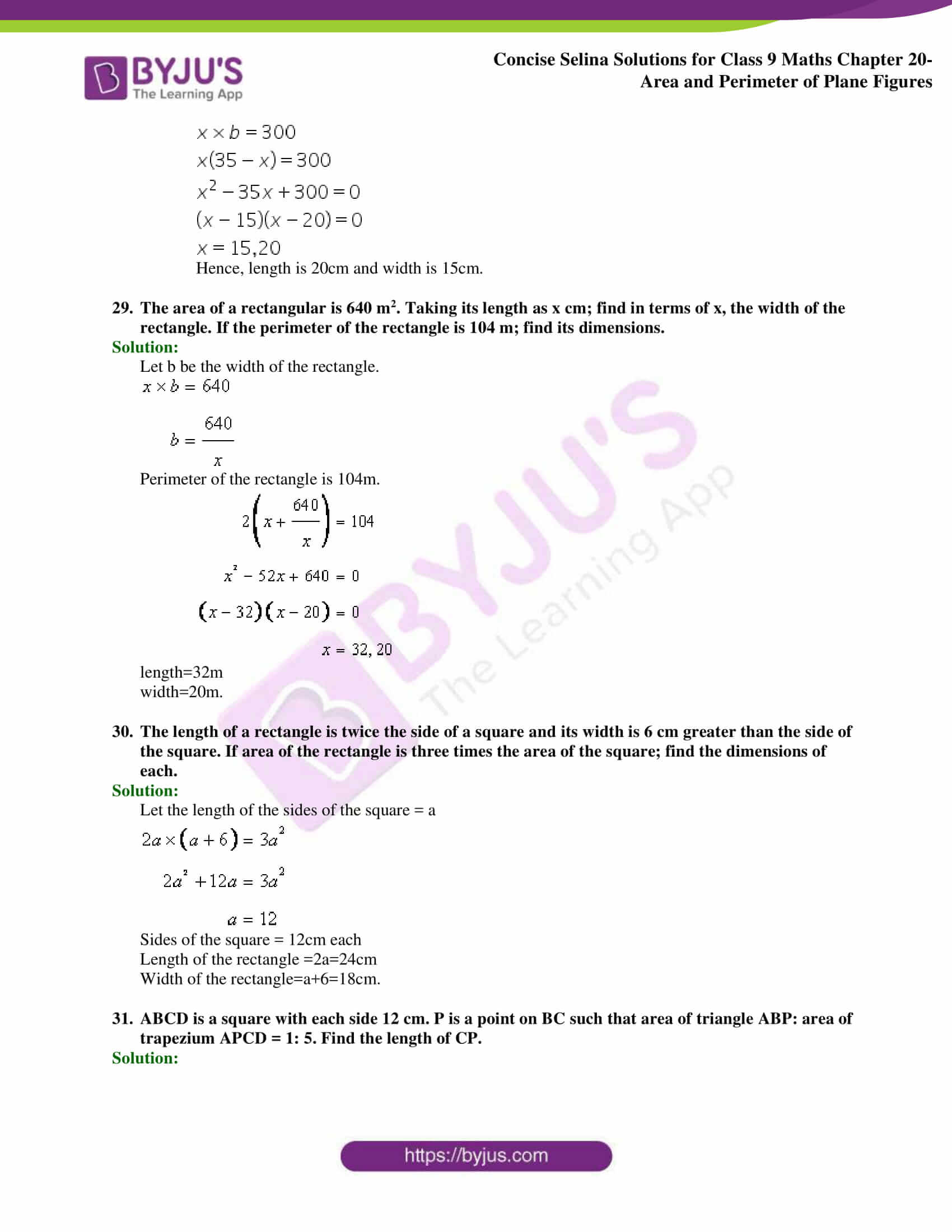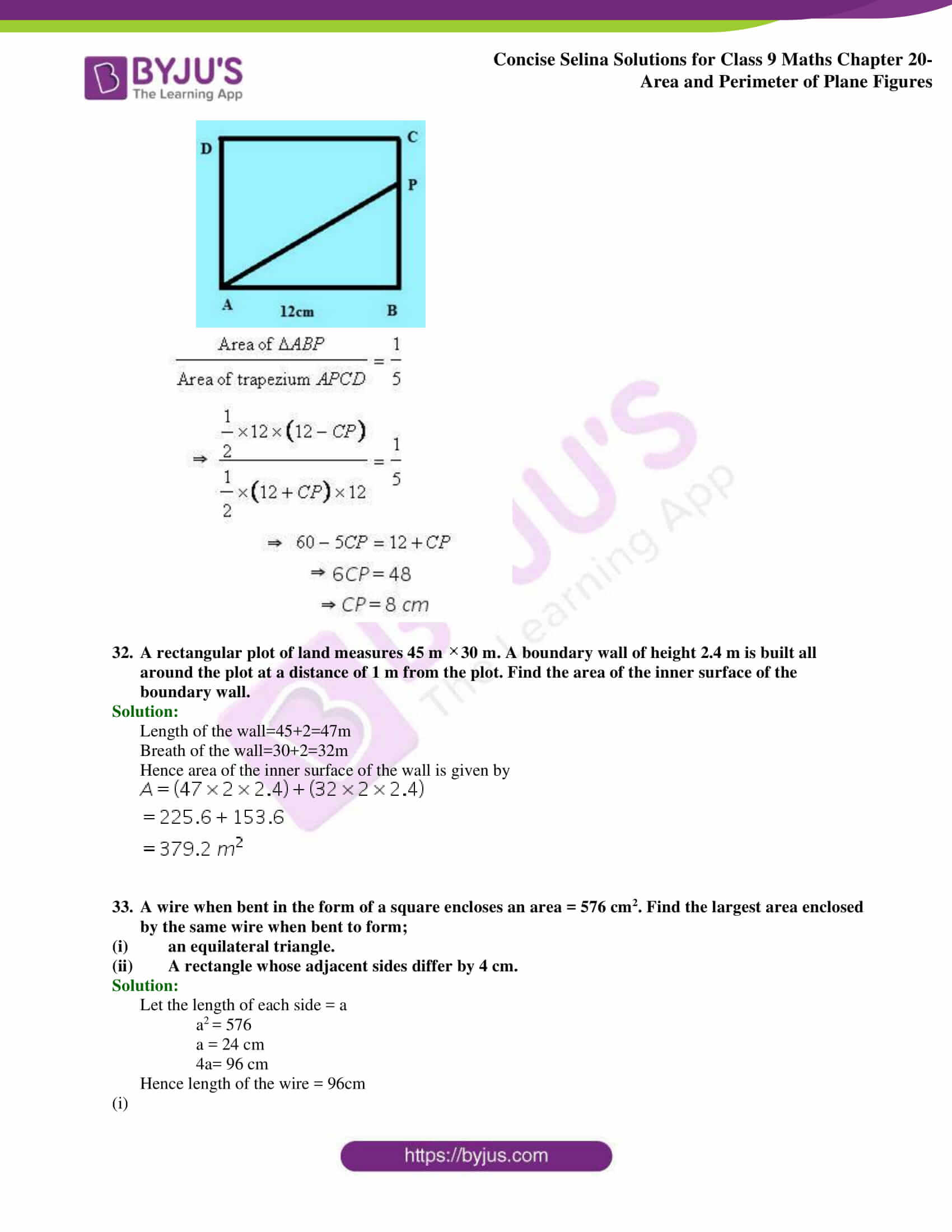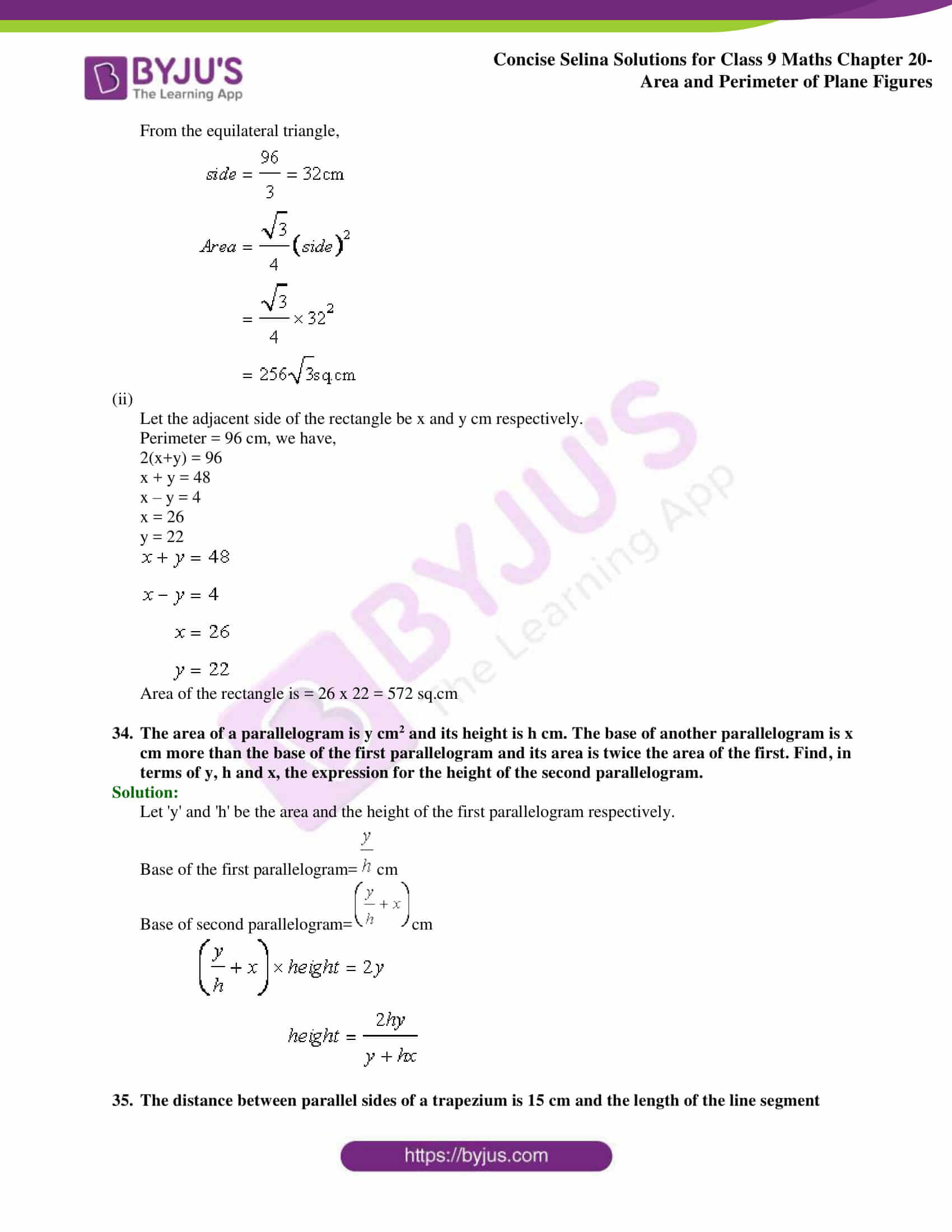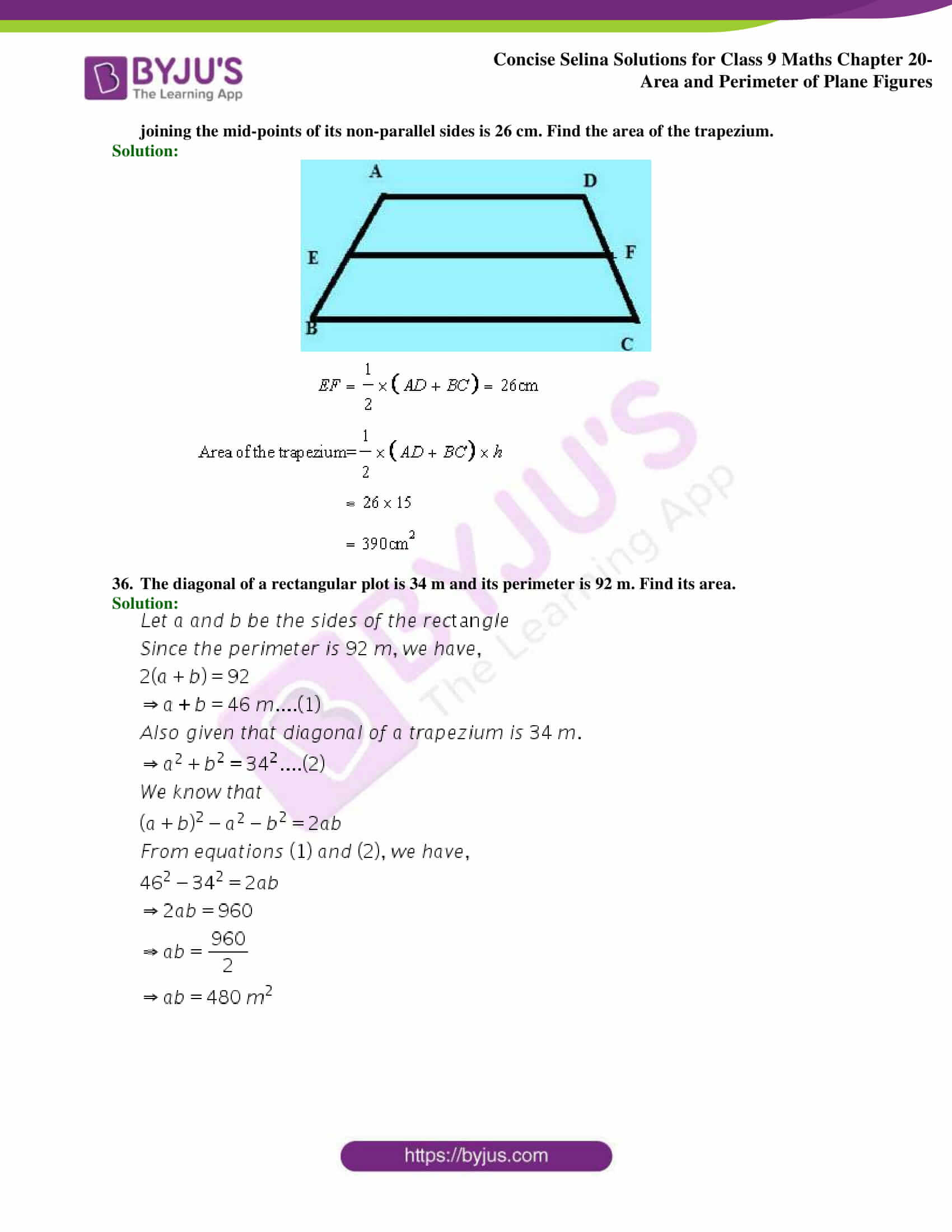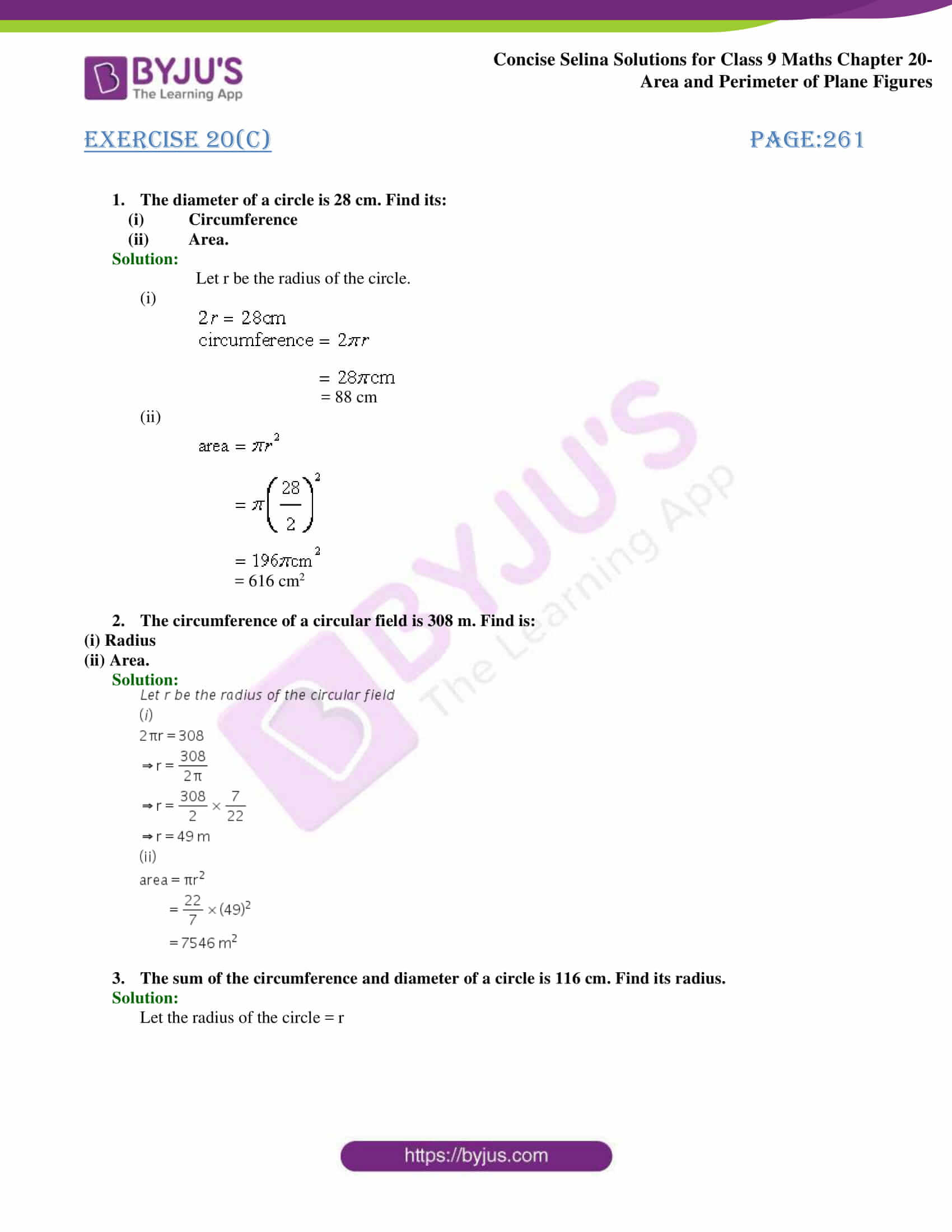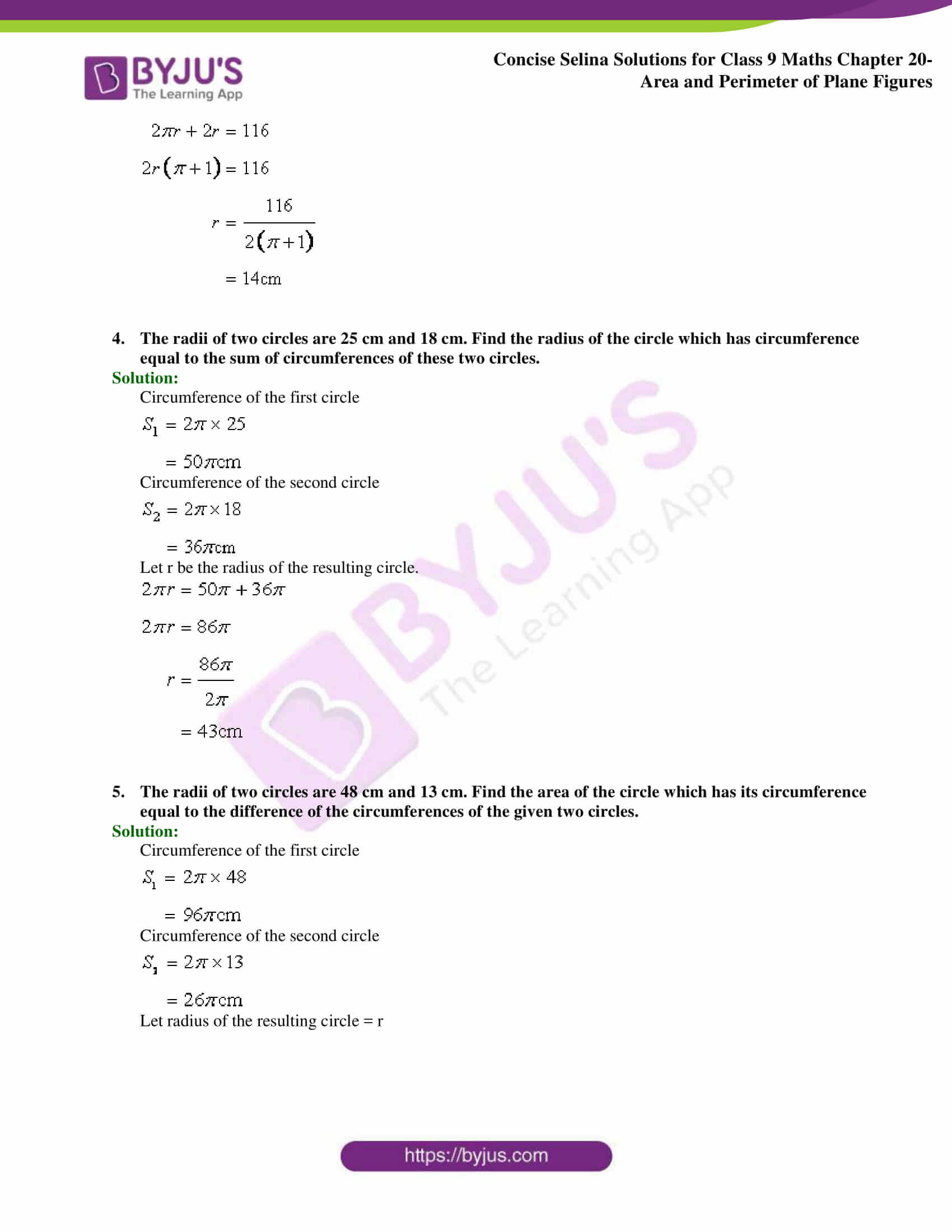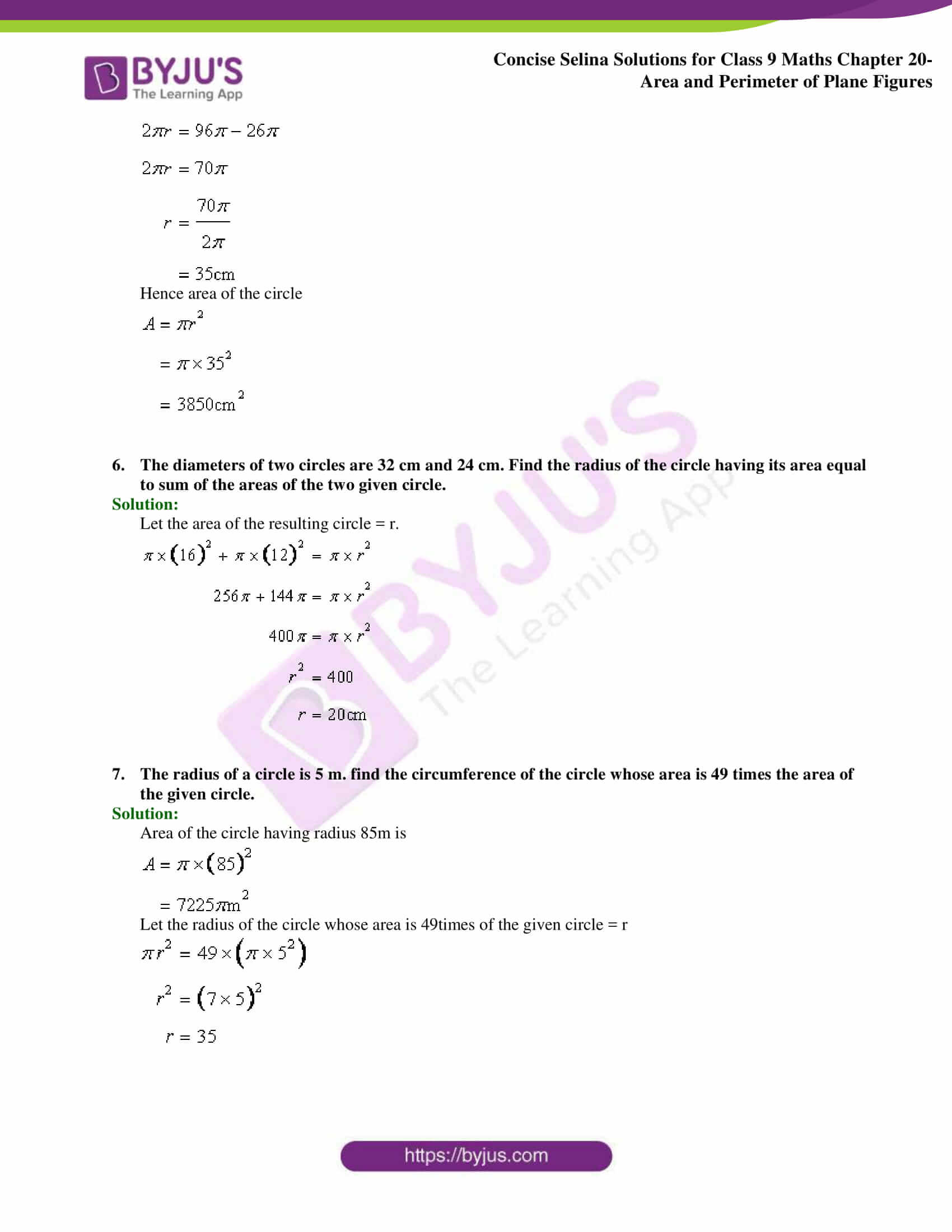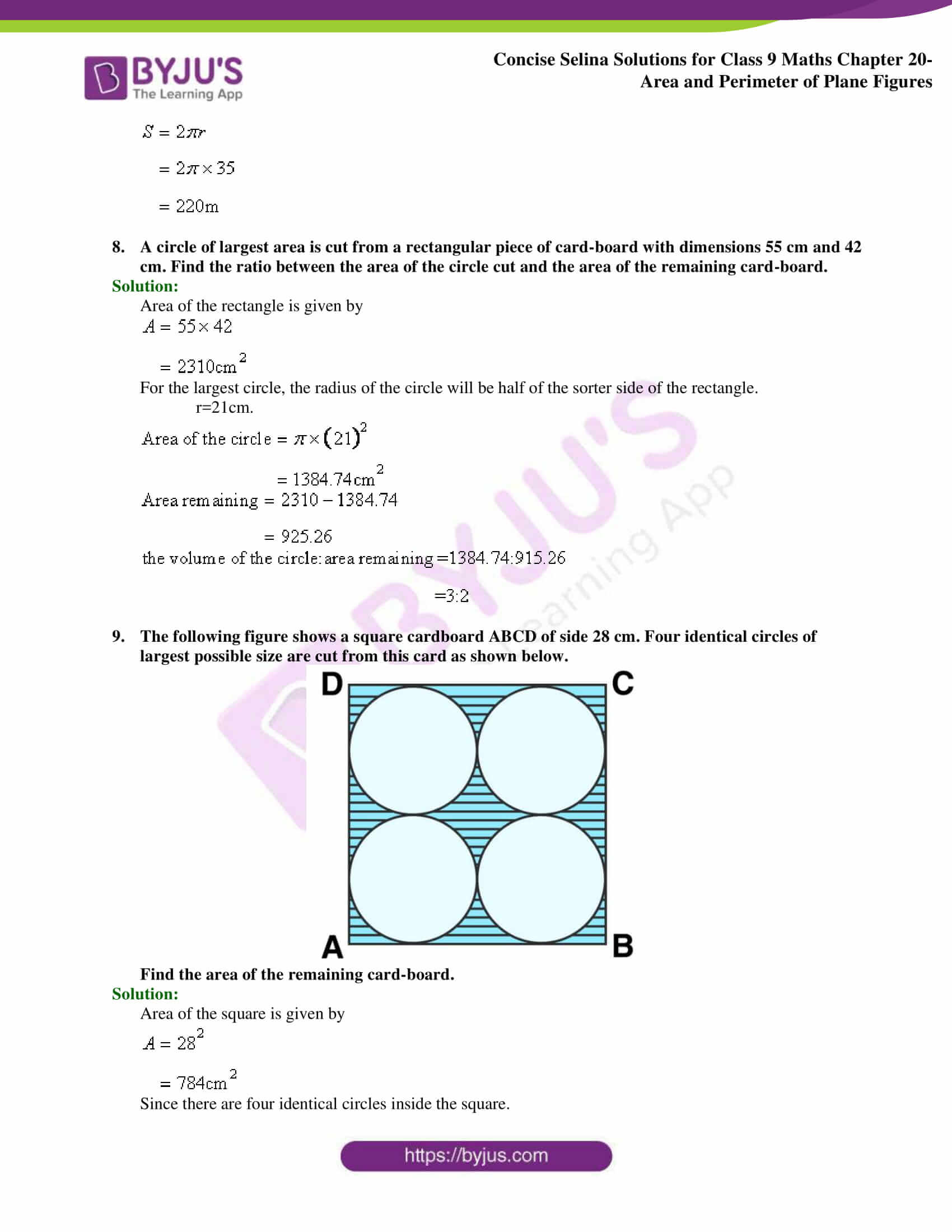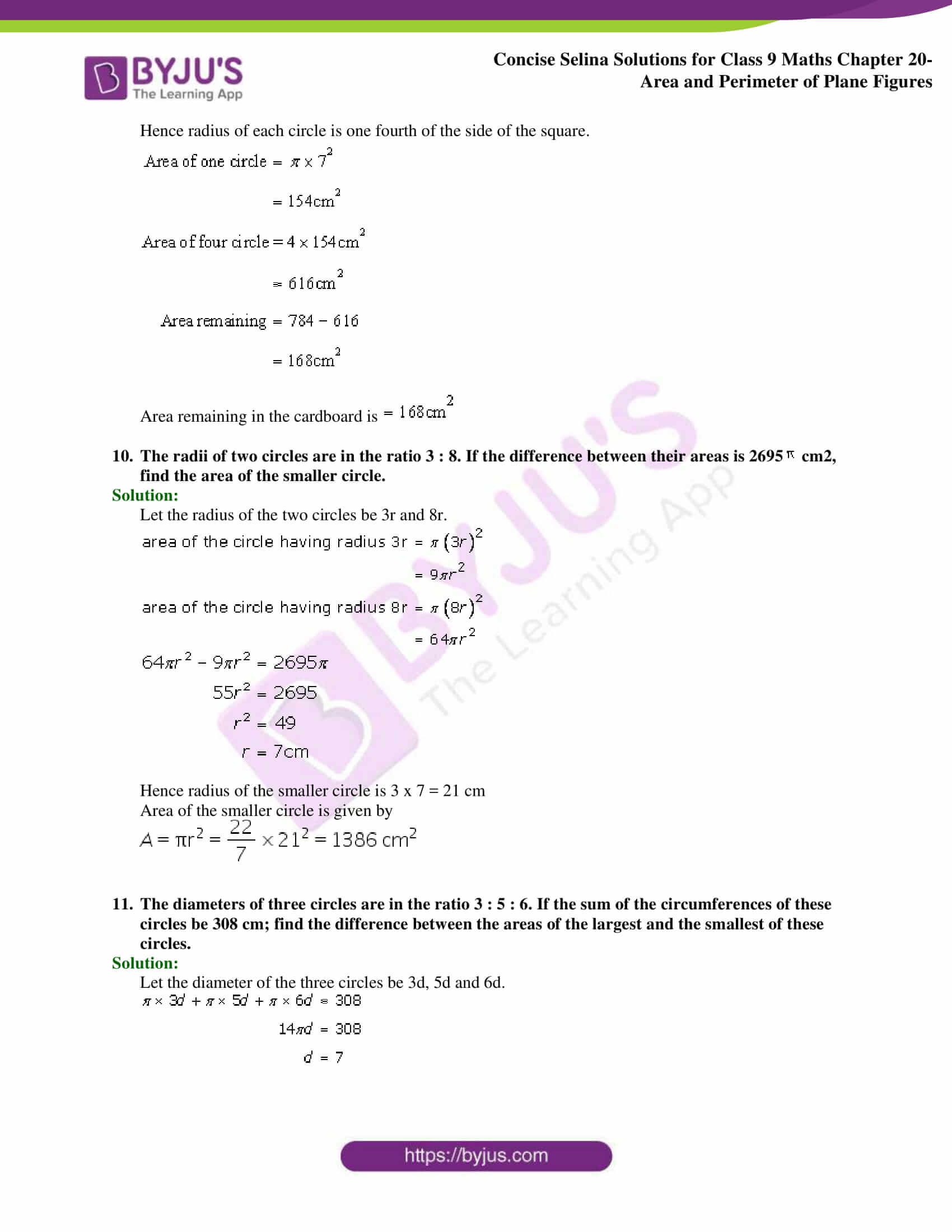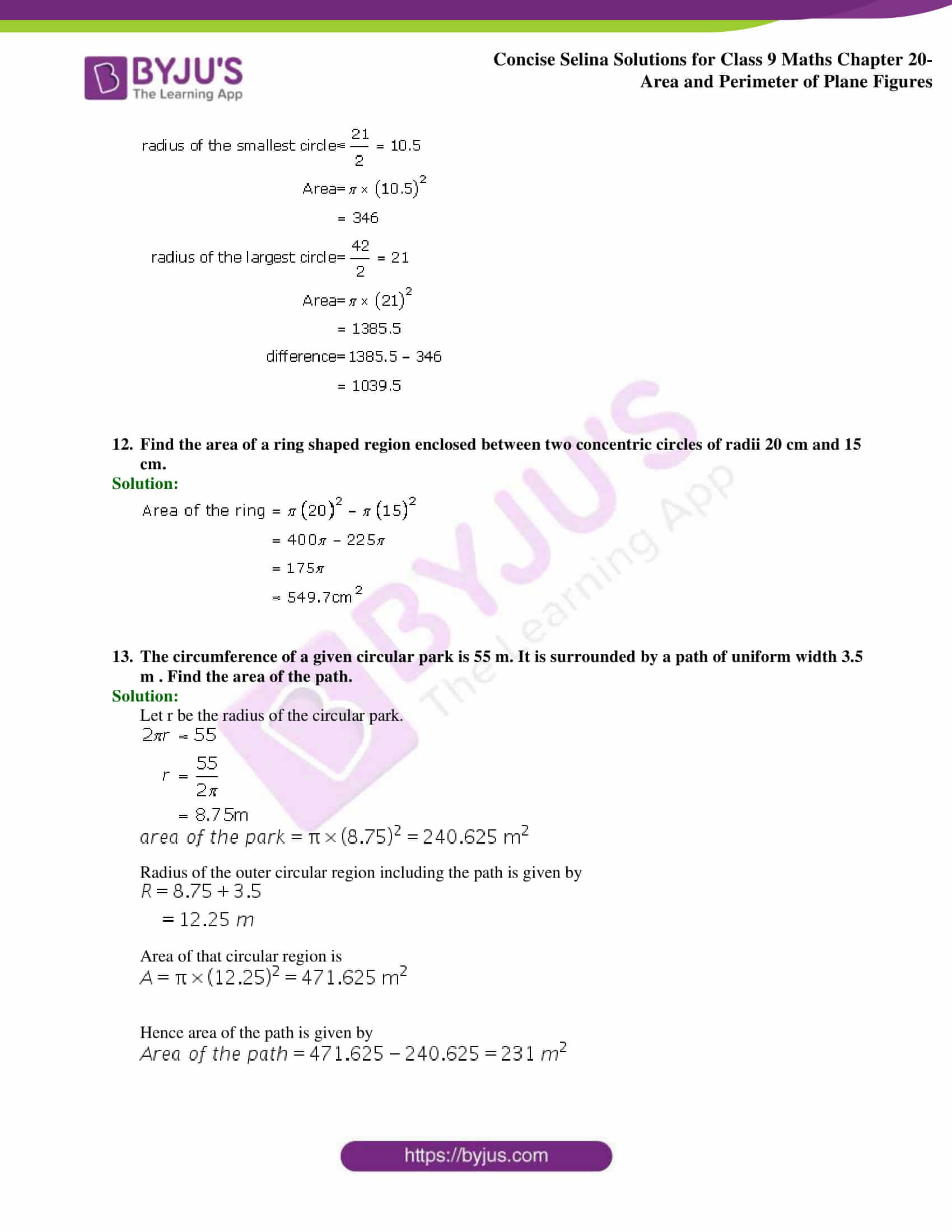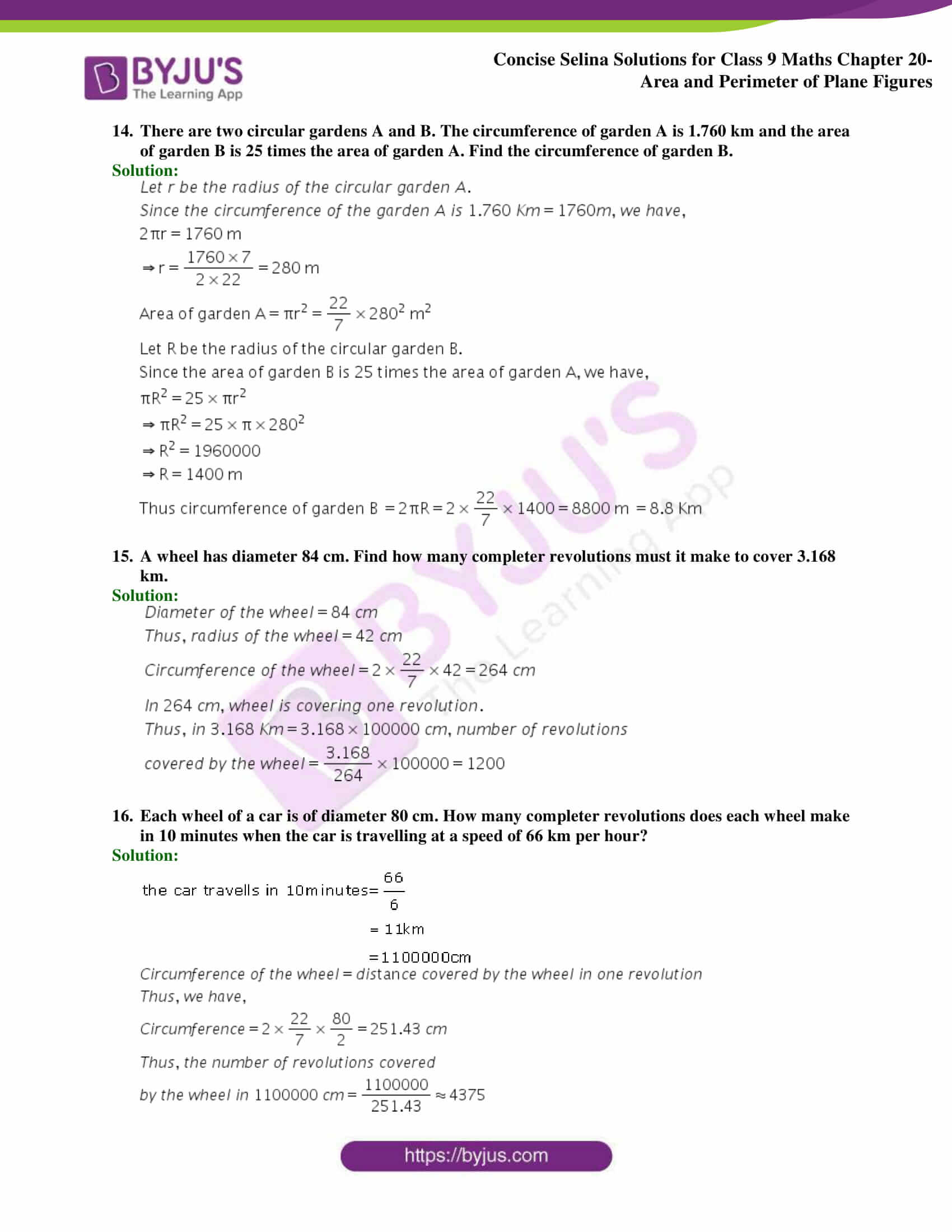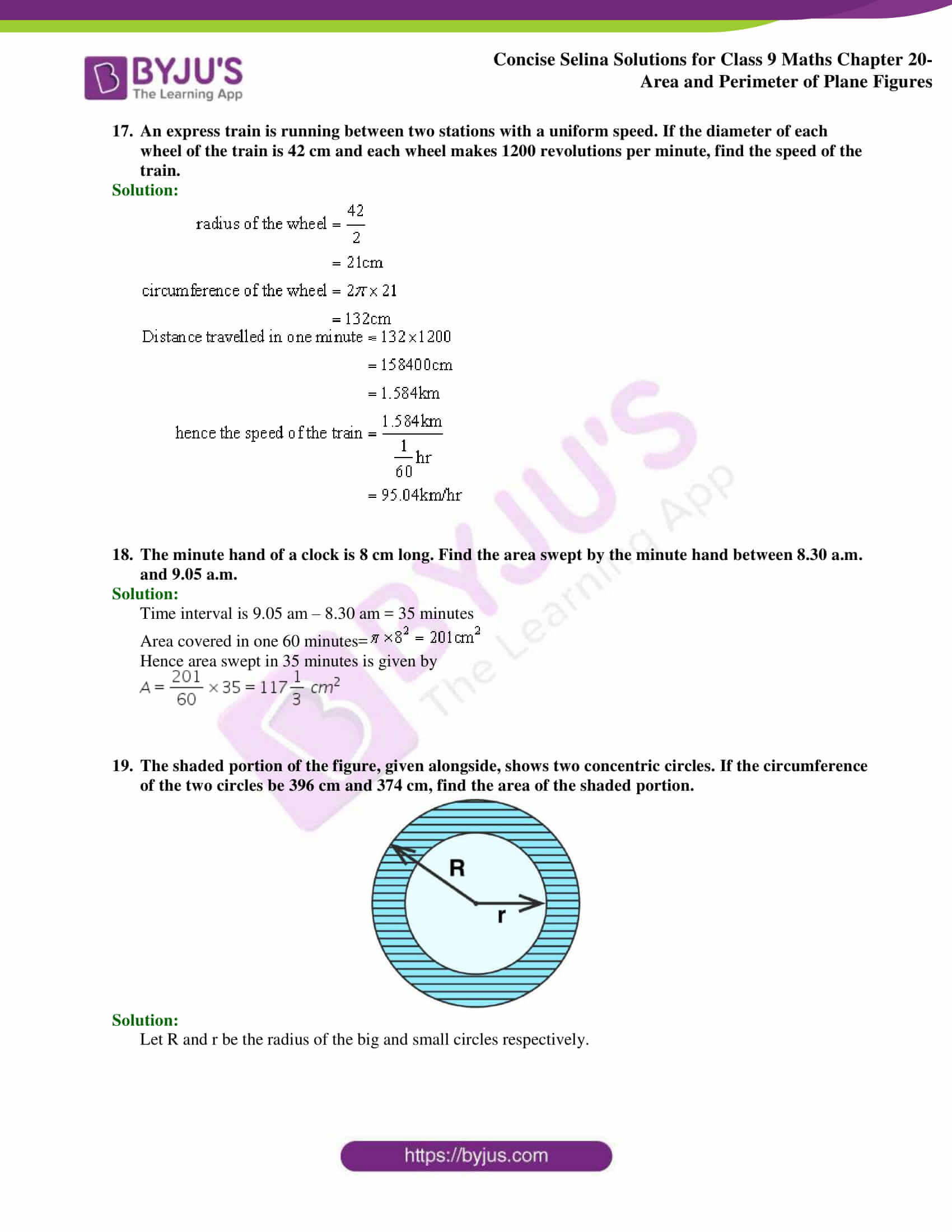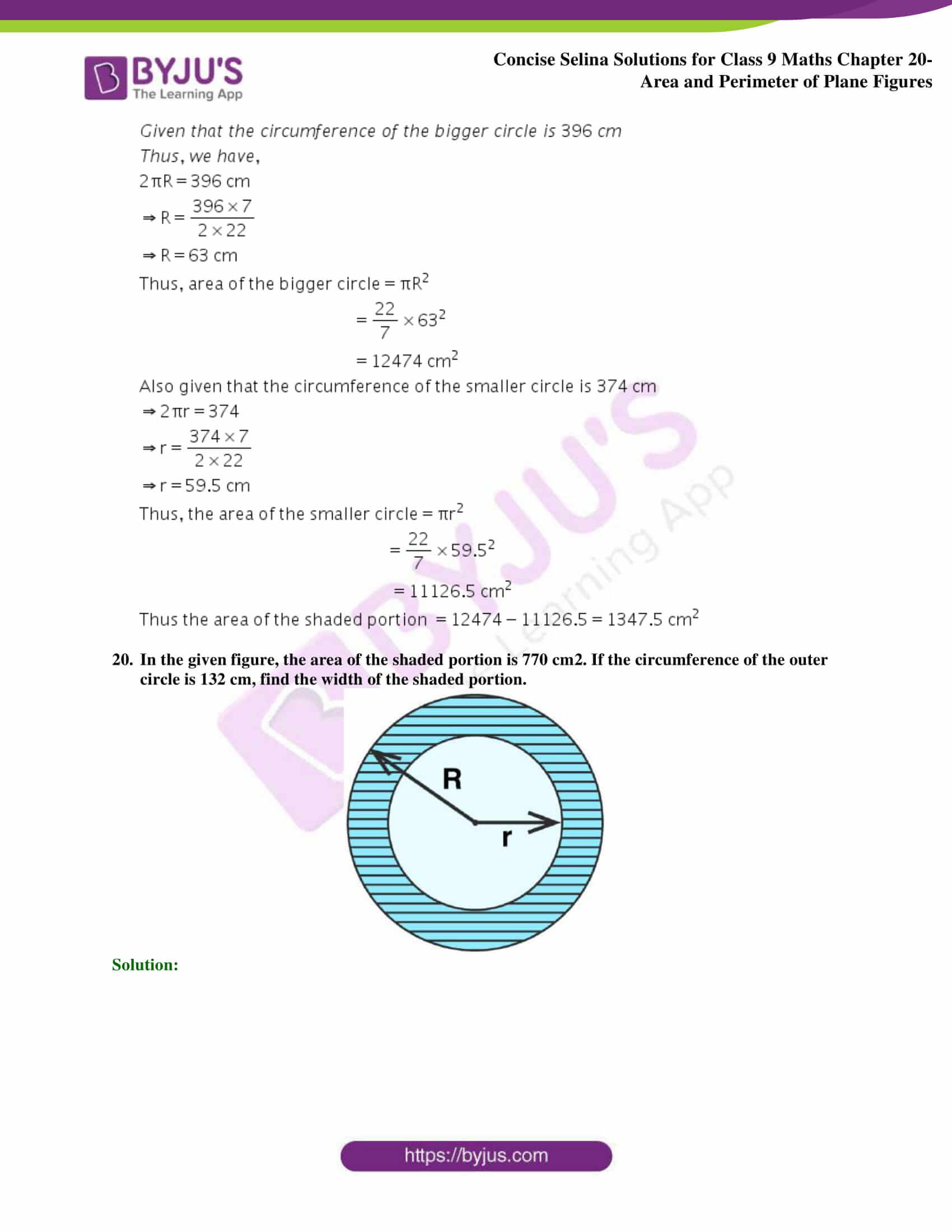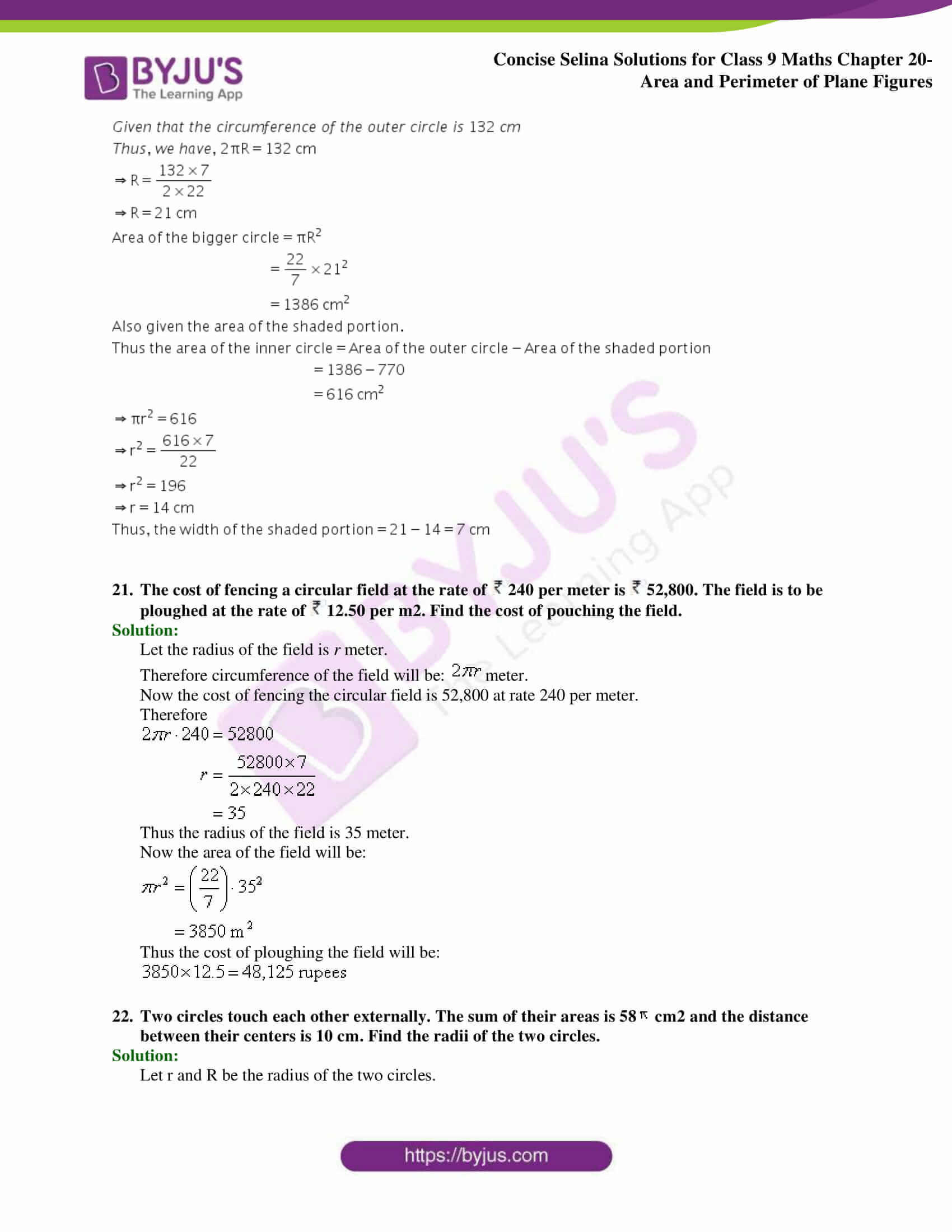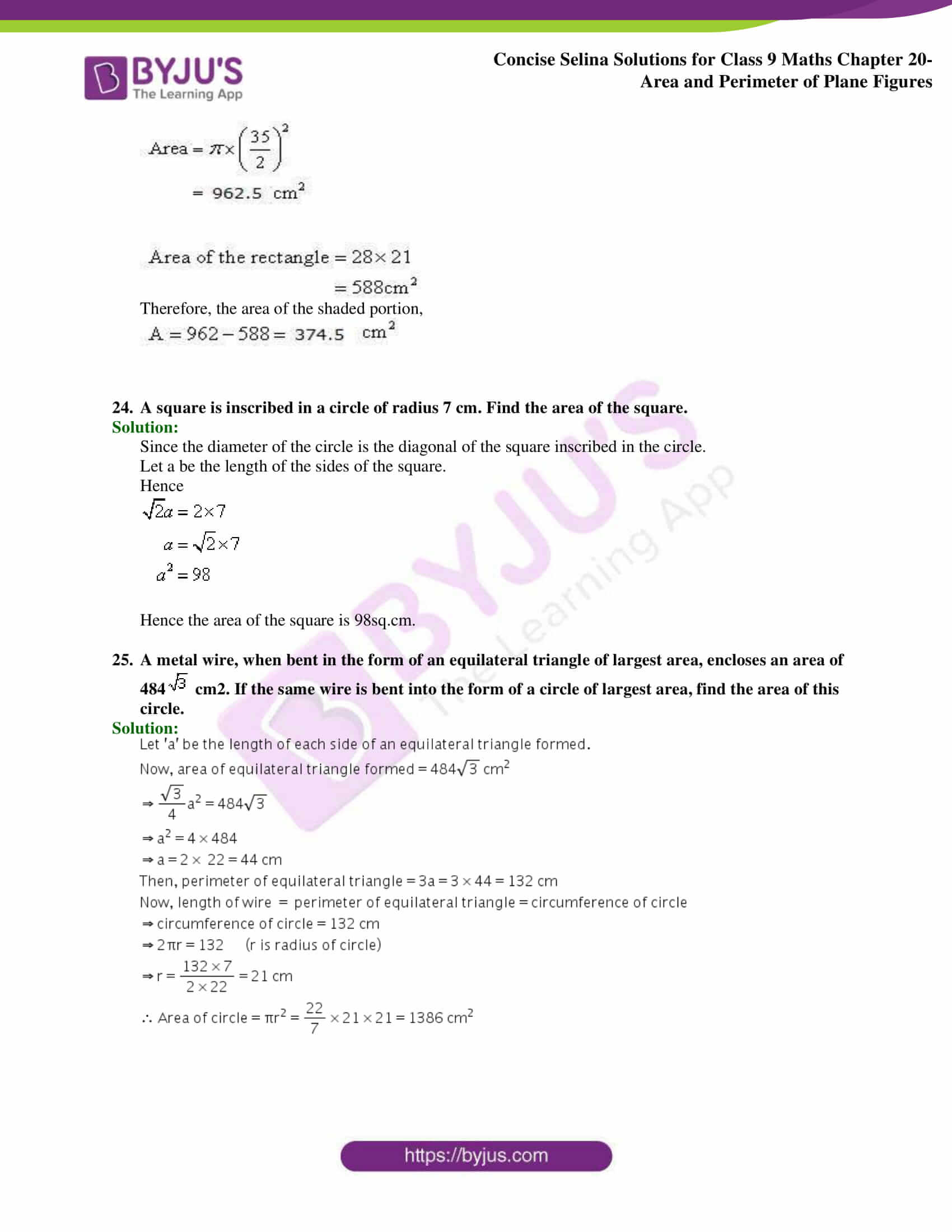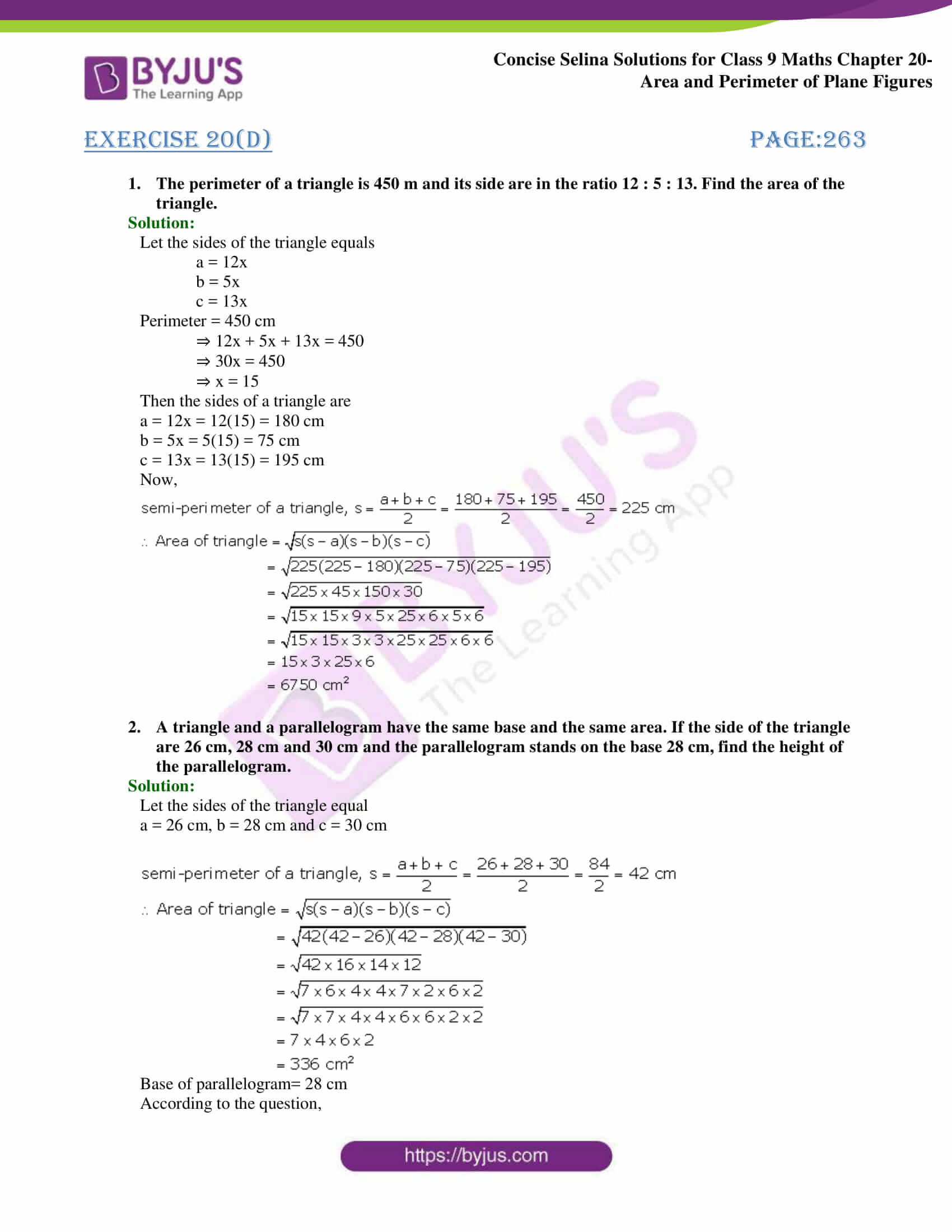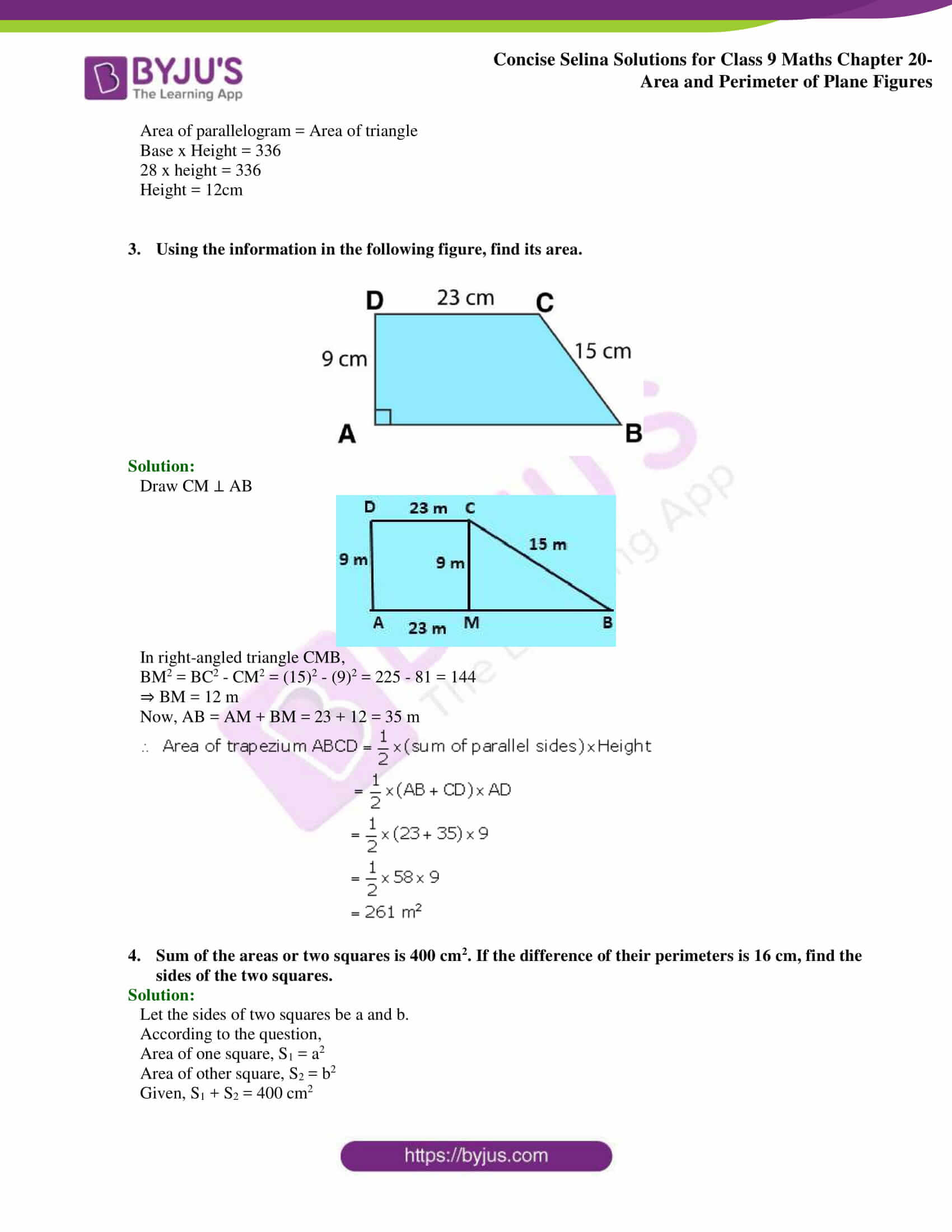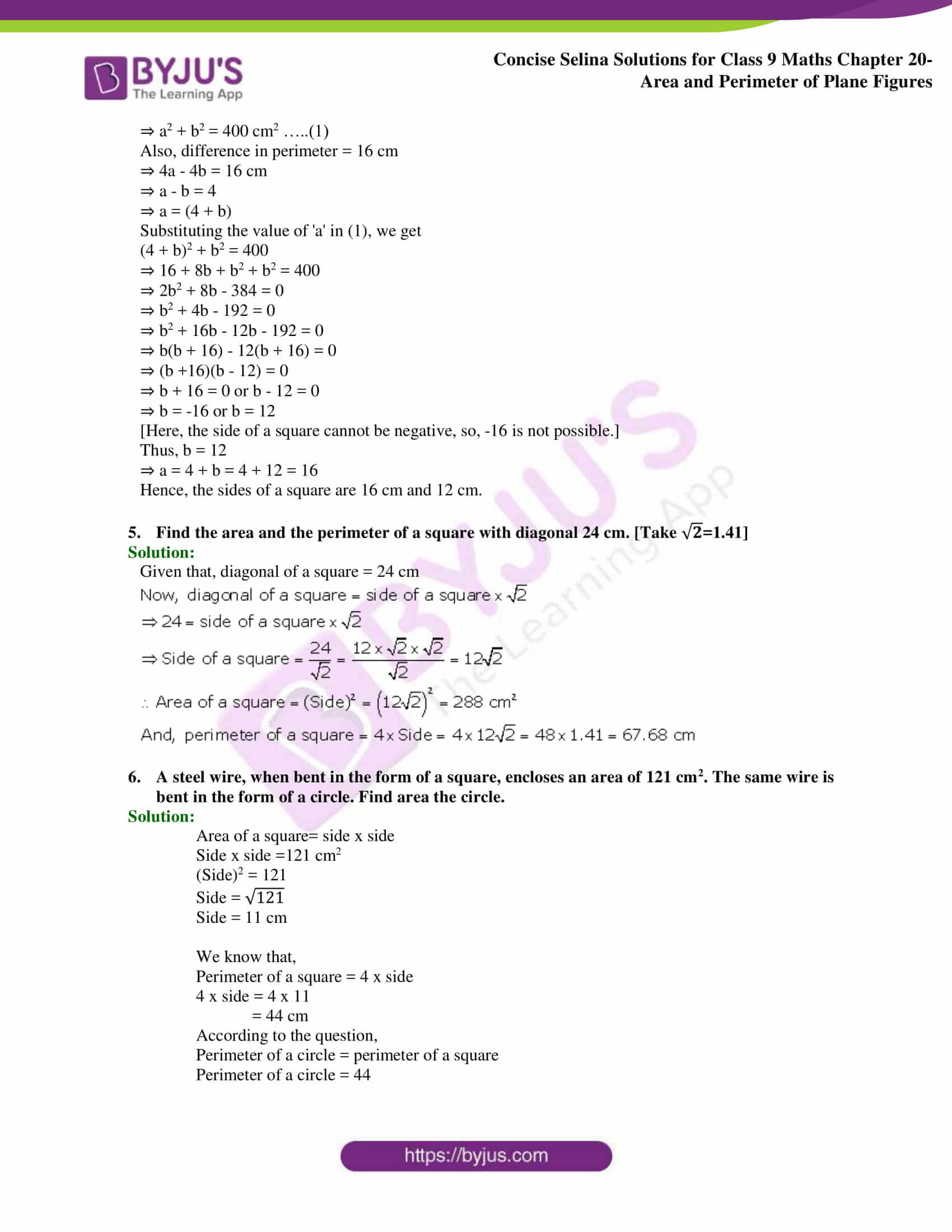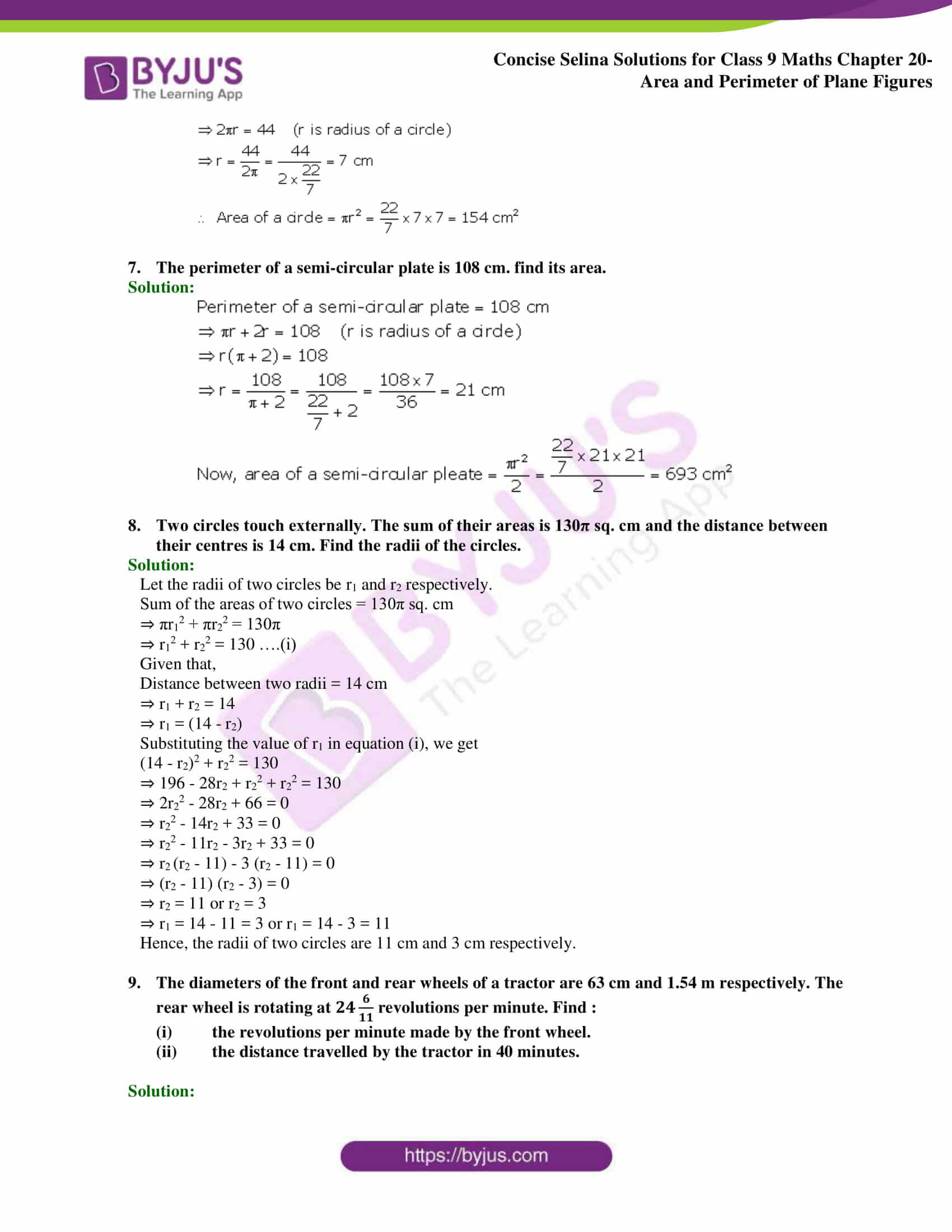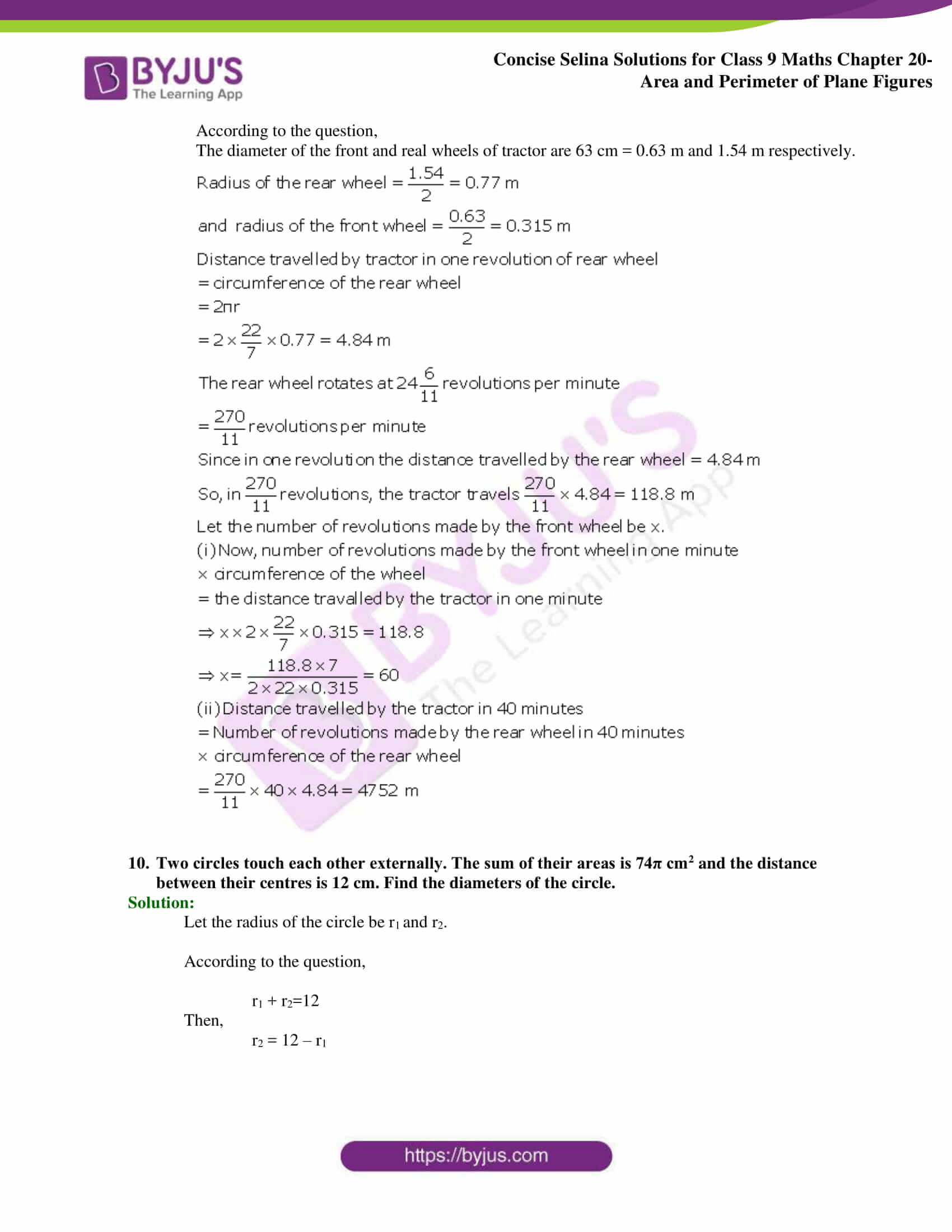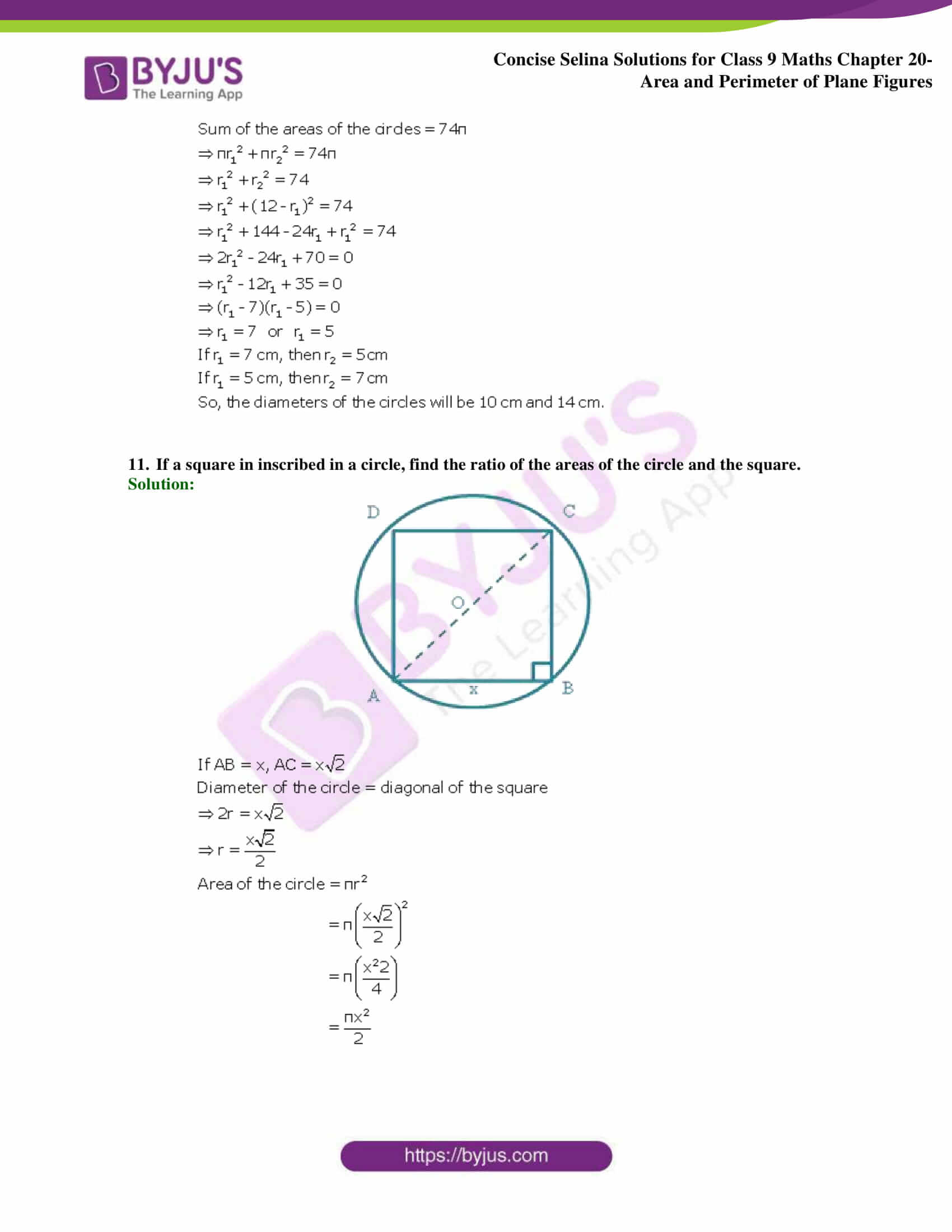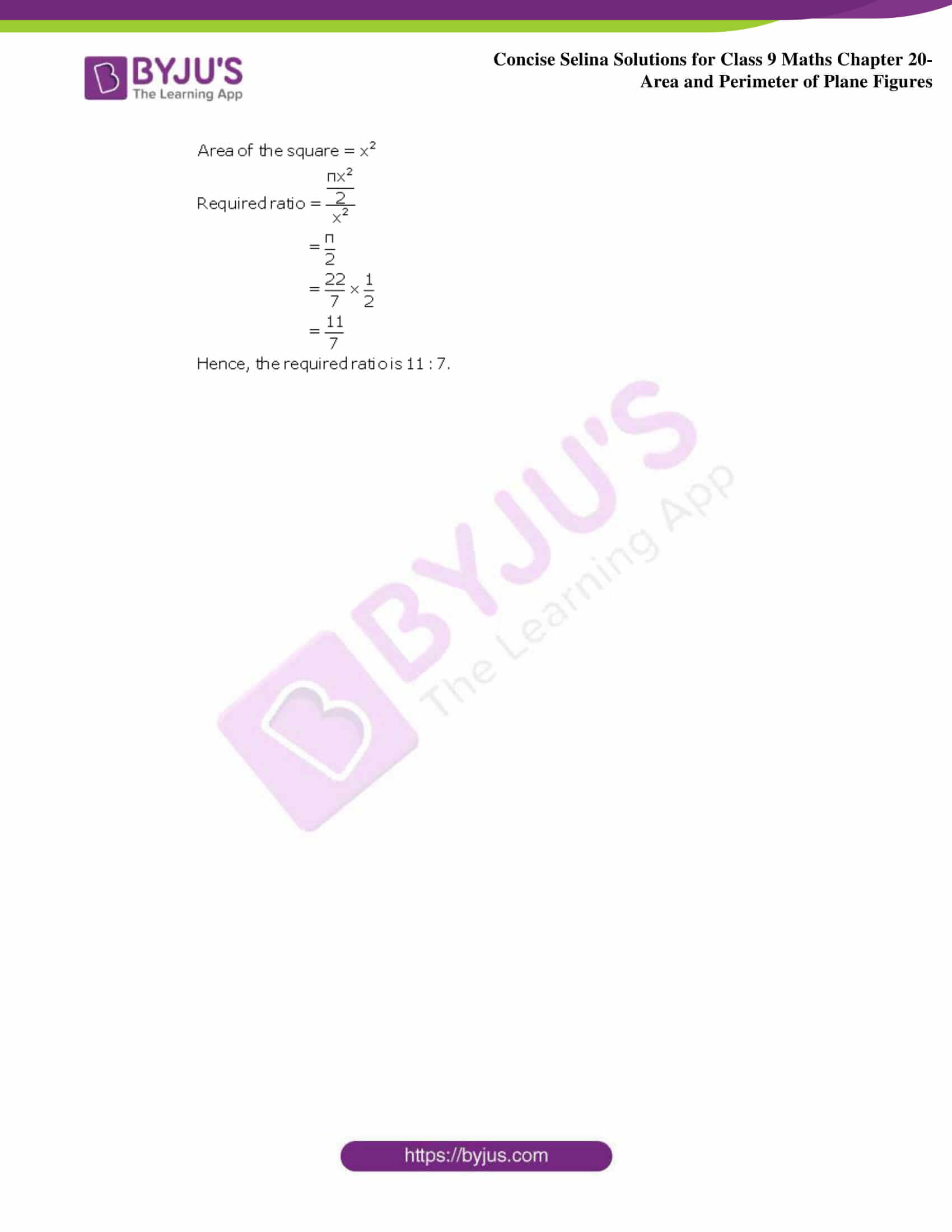## Selina Solutions for Class 9 Maths Chapter 20 – Area and Perimeter of Plane Figures

The Chapter 20, Area and Perimeter of Plane Figures, has 4 exercises and the Selina Solutions given here contains the answers to all the questions present in these exercises. Let us have a look at some of the topics that are being discussed in this chapter.

20.1 Introduction

20.2 Area and perimeter of triangles.

20.3 Some special types of triangles

20.4 Area and Perimeter of quadrilaterals

20.5 Some special types of quadrilaterals

20.6 Circumference of a circle

20.7 Area of a circle

## Selina Solutions for Class 9 Maths Chapter 20-Area and Perimeter of Plane Figures

The perimeter of a plane figure is the length of its boundary. On the other hand, the area of a plane figure is the measure of the surface enclosed by its boundary. Chapter 20 of class 9 gives the students an overview of the Area as well as the Perimeter of Plane Figures. Read and learn Chapter 20 of Selina textbook to learn more about Area and Perimeter of Plane Figures, along with the concepts covered in it. Solve the Selina Solutions for Class 9 effectively to score high in the examination.